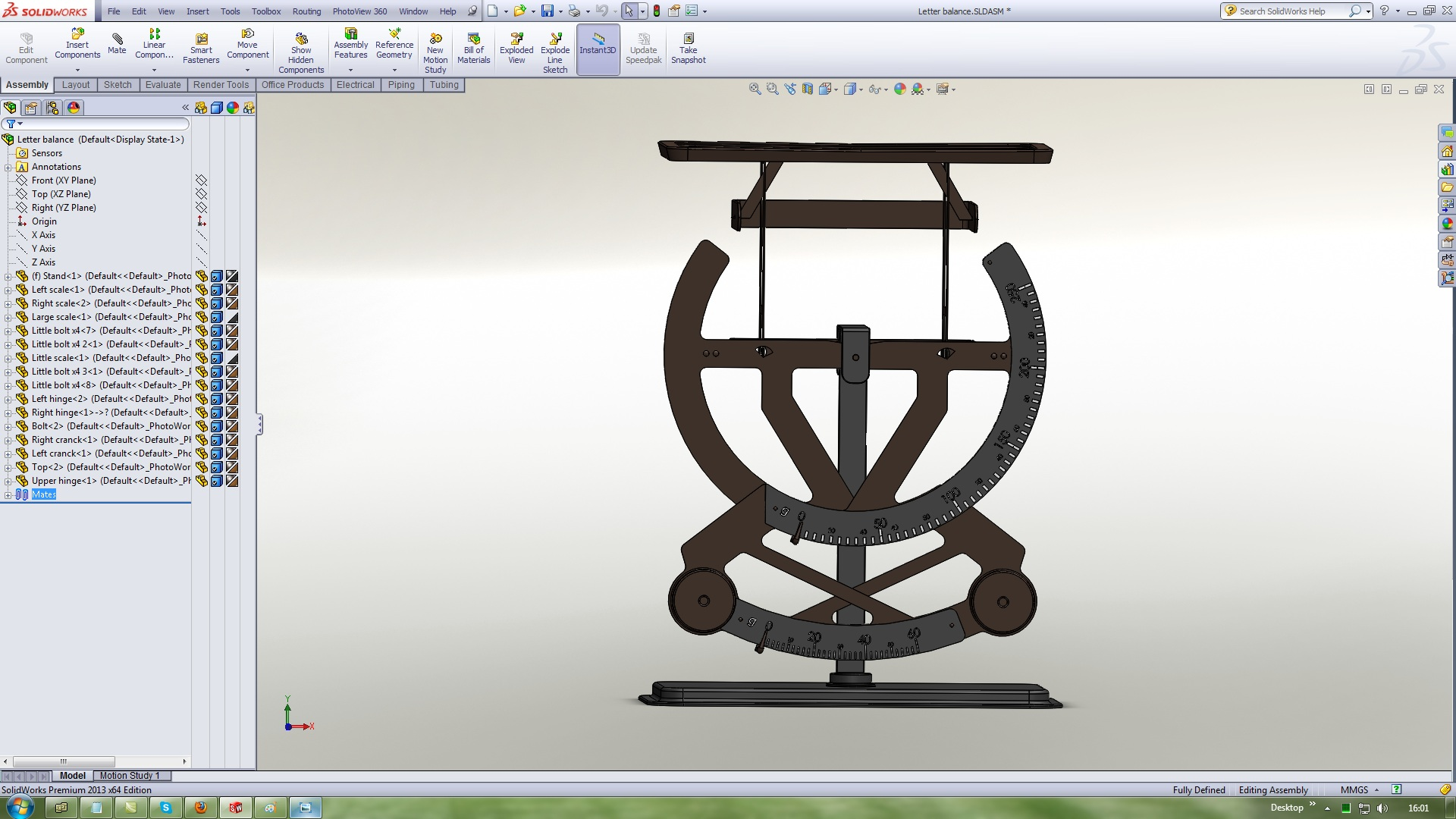# How to model Letter Balance in Solidwoks - Assembly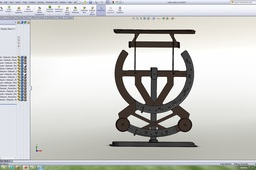Letter Balance - Fully Defined

Before start the assembly, copy Little bolt four times with diferent names

1. ### Step 1:

Create a new assembly and insert the Stand on this position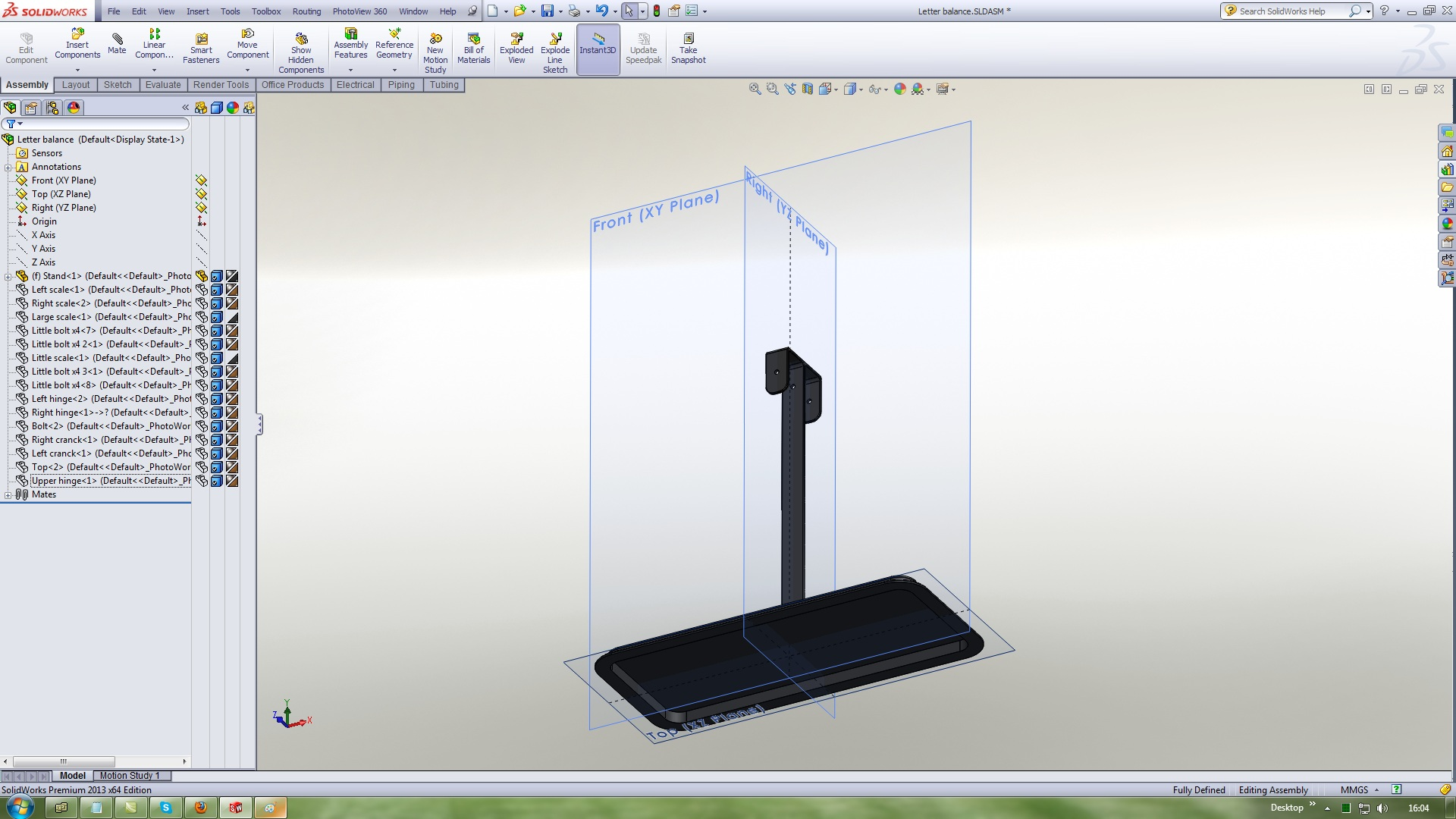2. ### Step 2:

Insert a Right scale
Use Mechanical mates Hinge with this references and values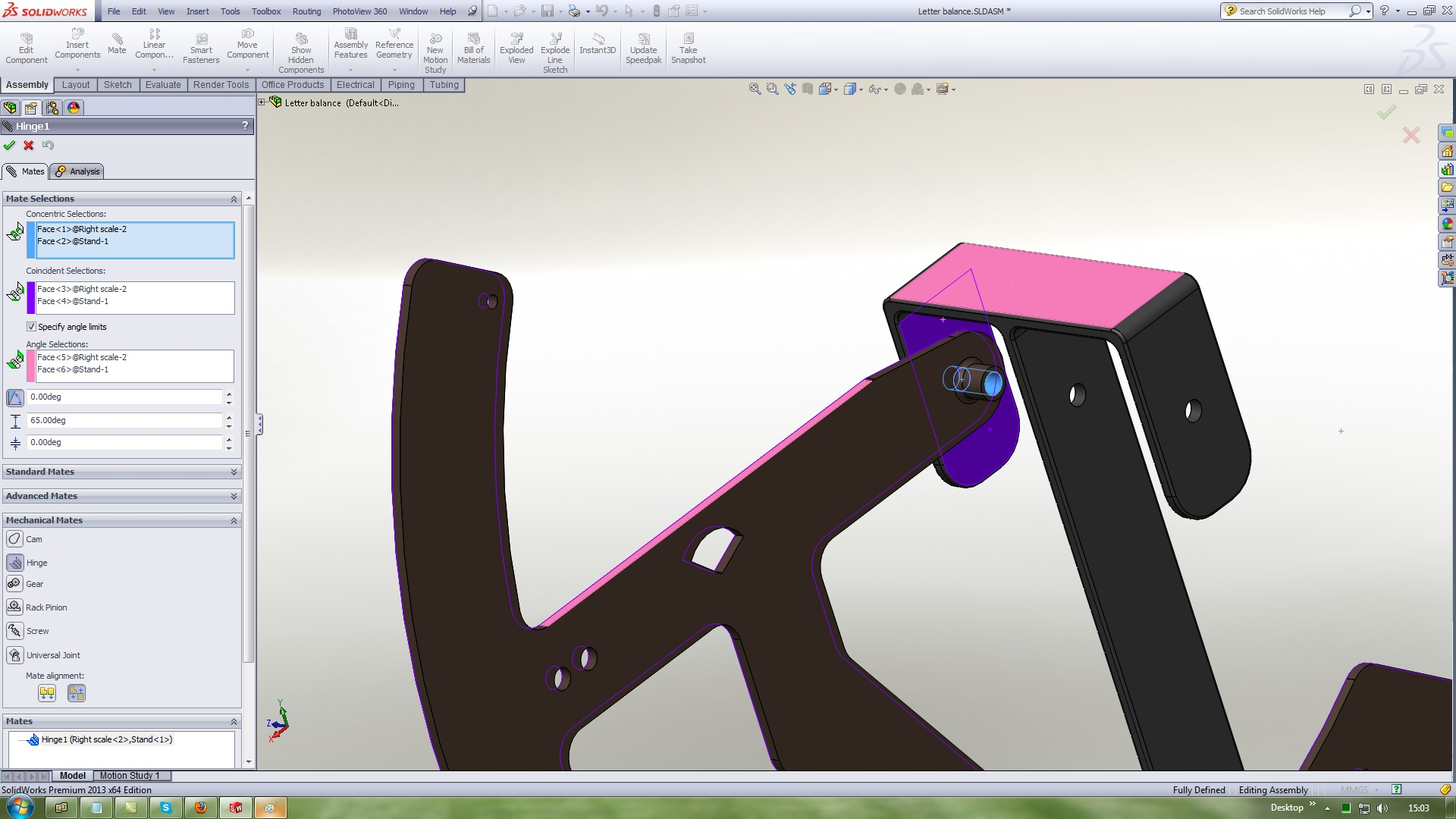3. ### Step 3:

Insert a Left scale
Use Mechanical mates Hinge with this references and values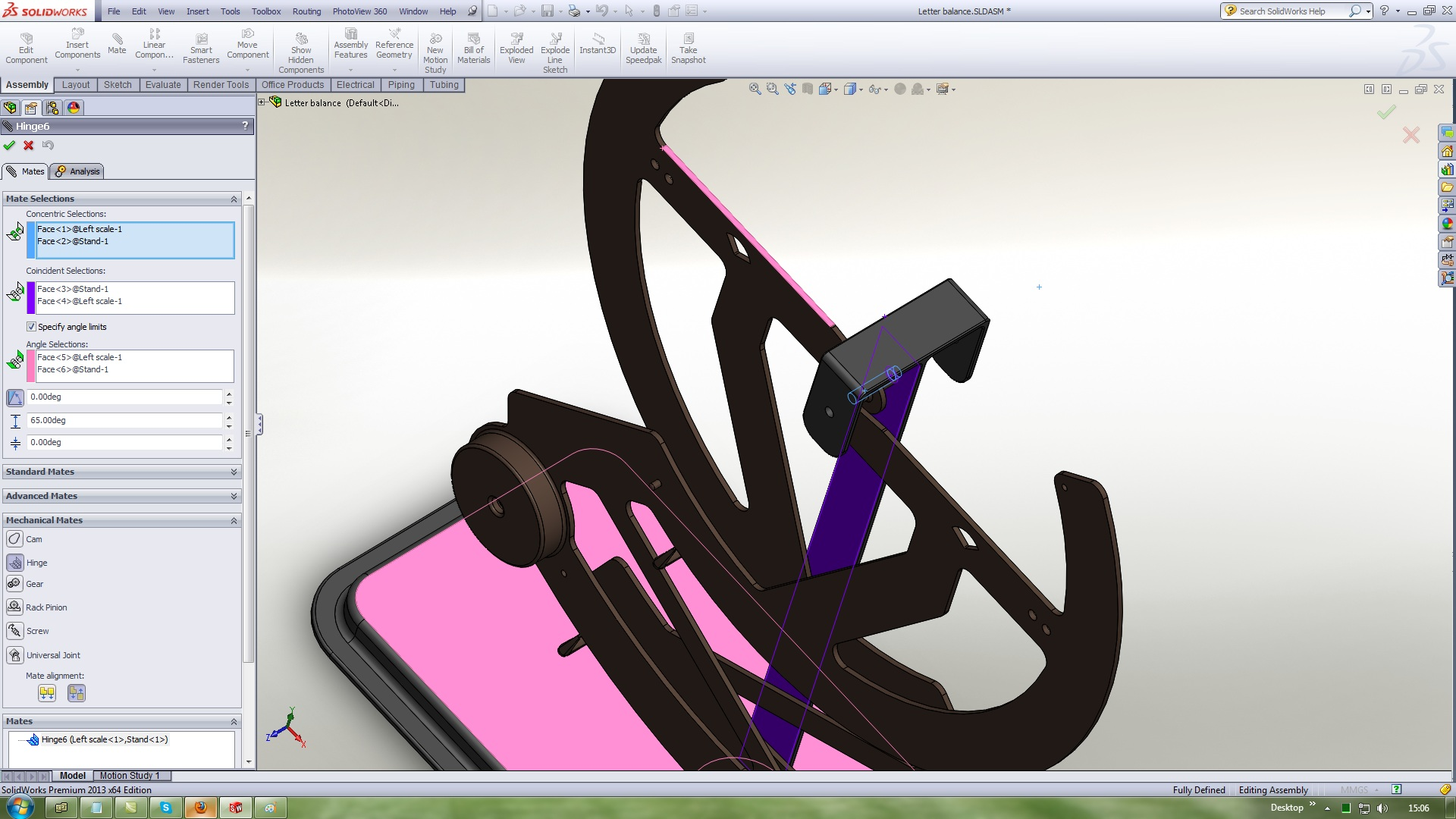4. ### Step 4:

Insert a Large scale
Use Standard mates Concentric with this references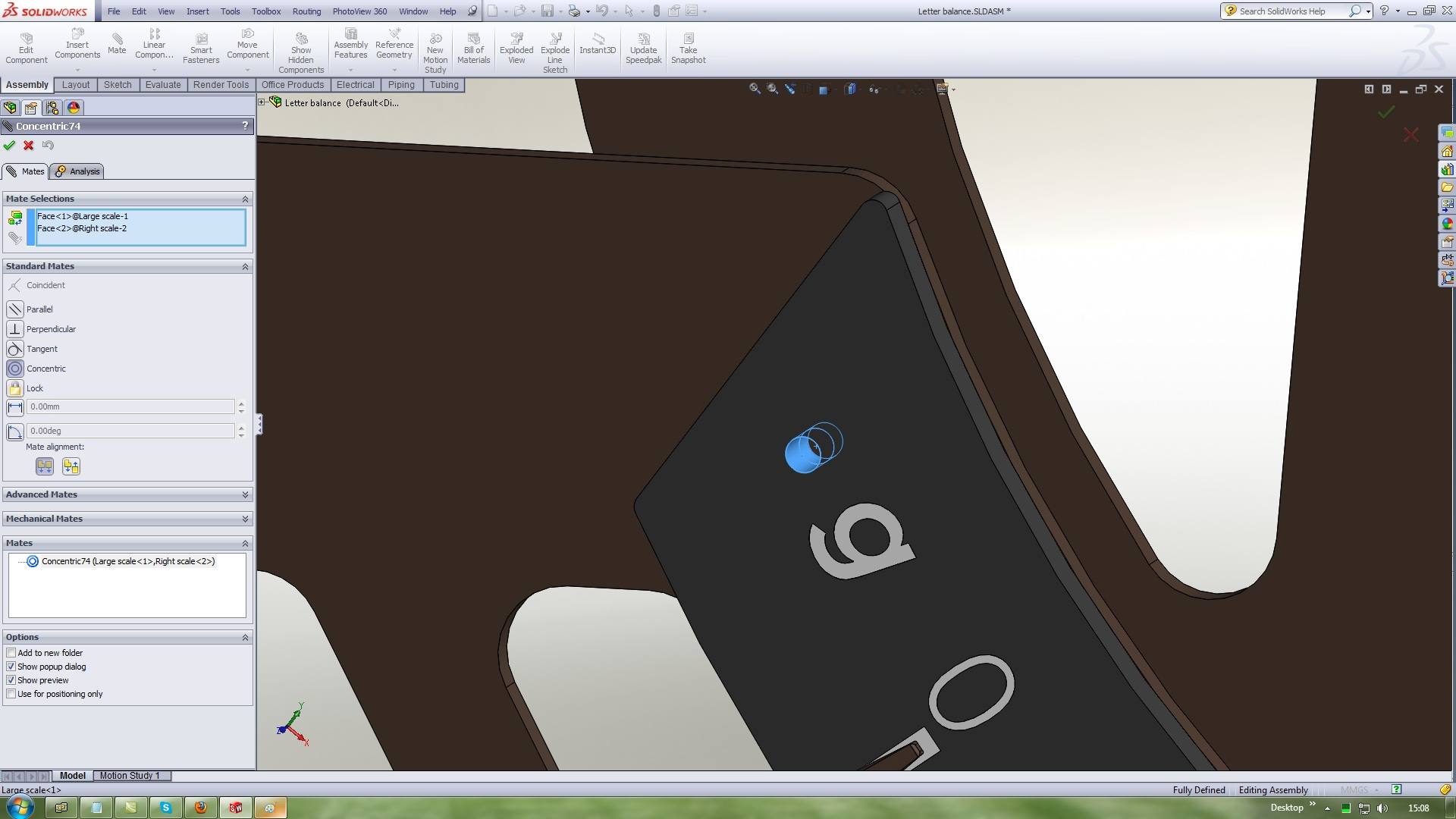5. ### Step 5:

Use Standard mates Coincident with this references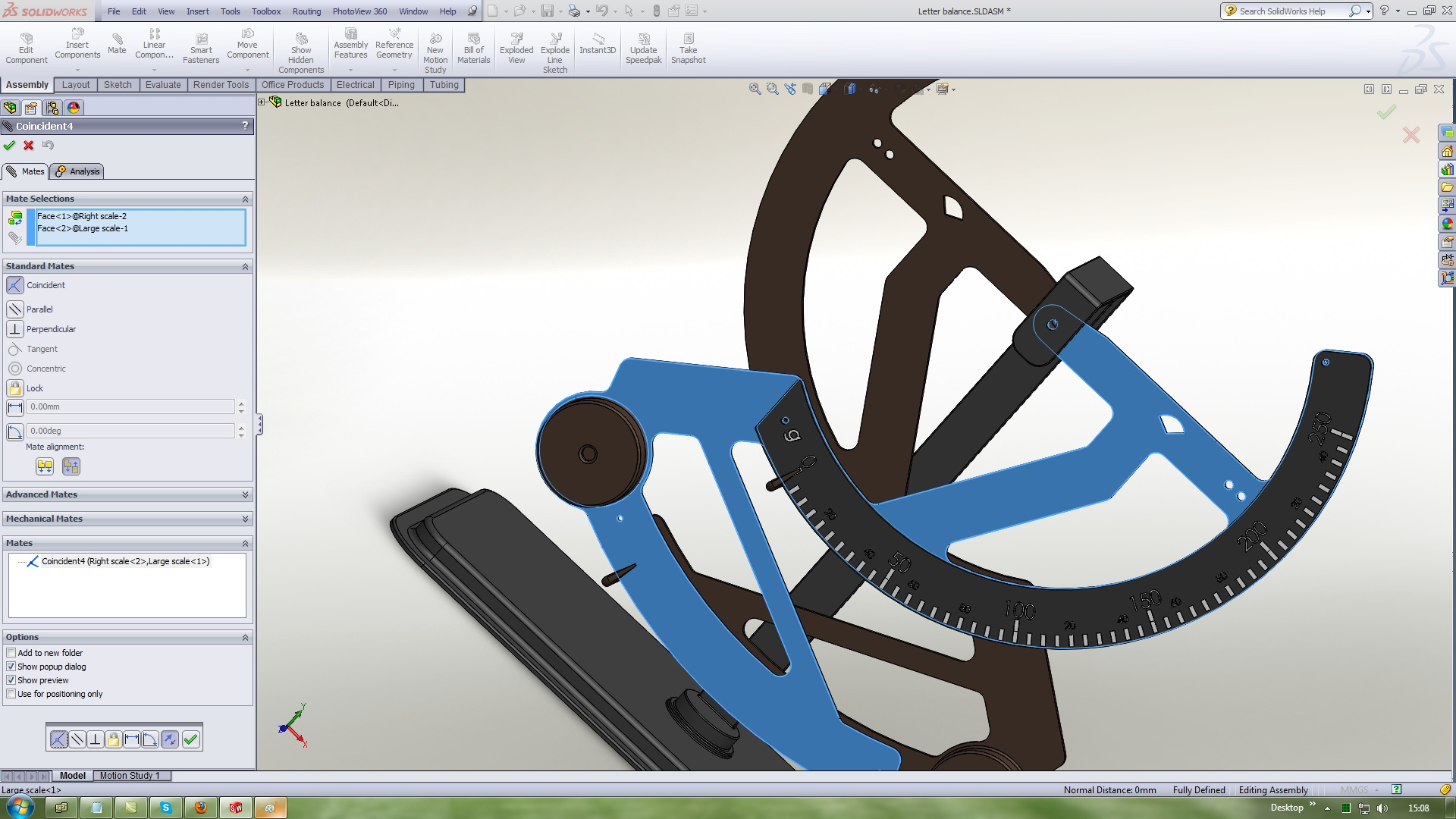6. ### Step 6:

Insert a Little bolt 1
Use Standard mates Concentric with this references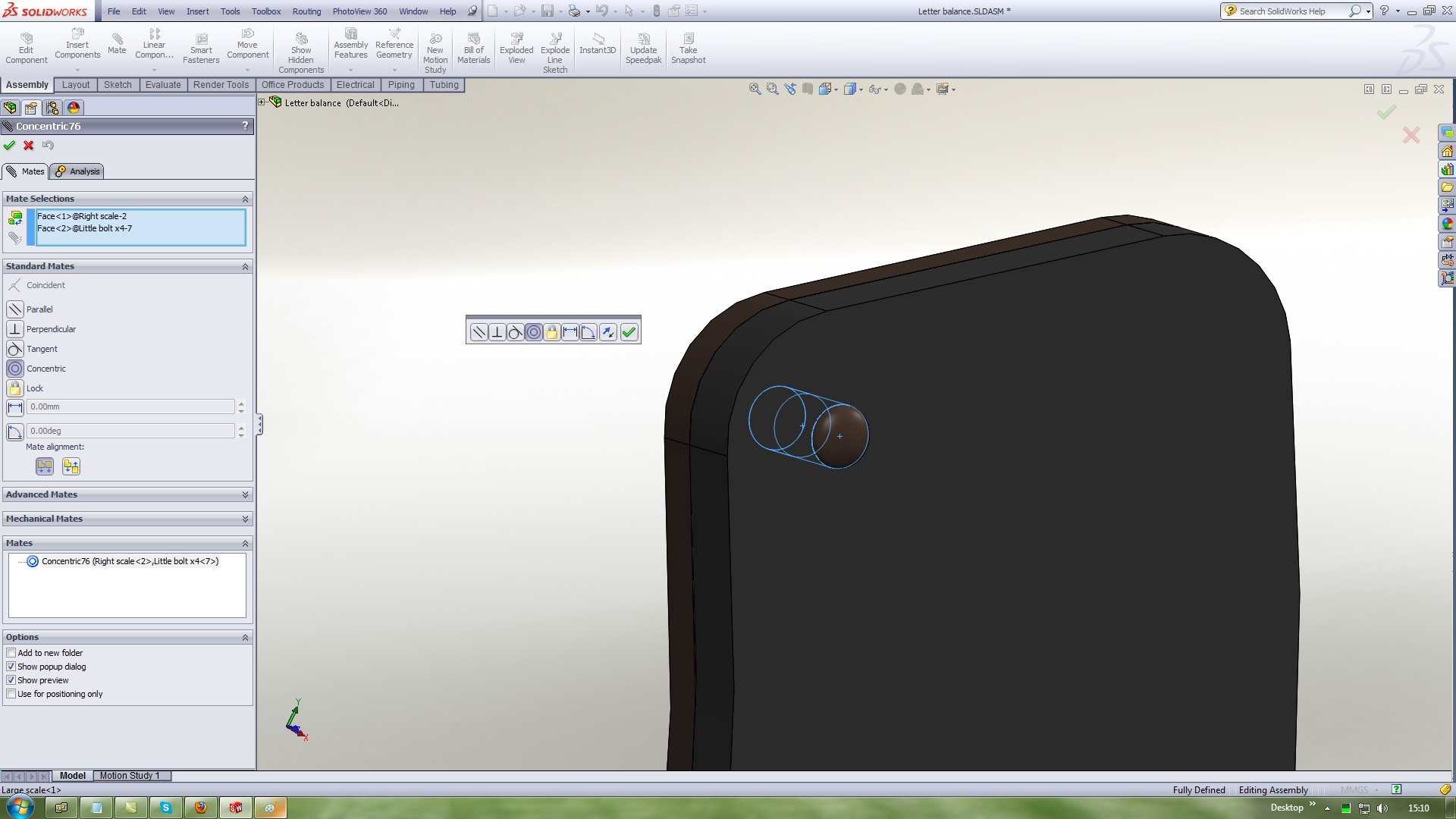7. ### Step 7:

Use Standard mates Coincident with this references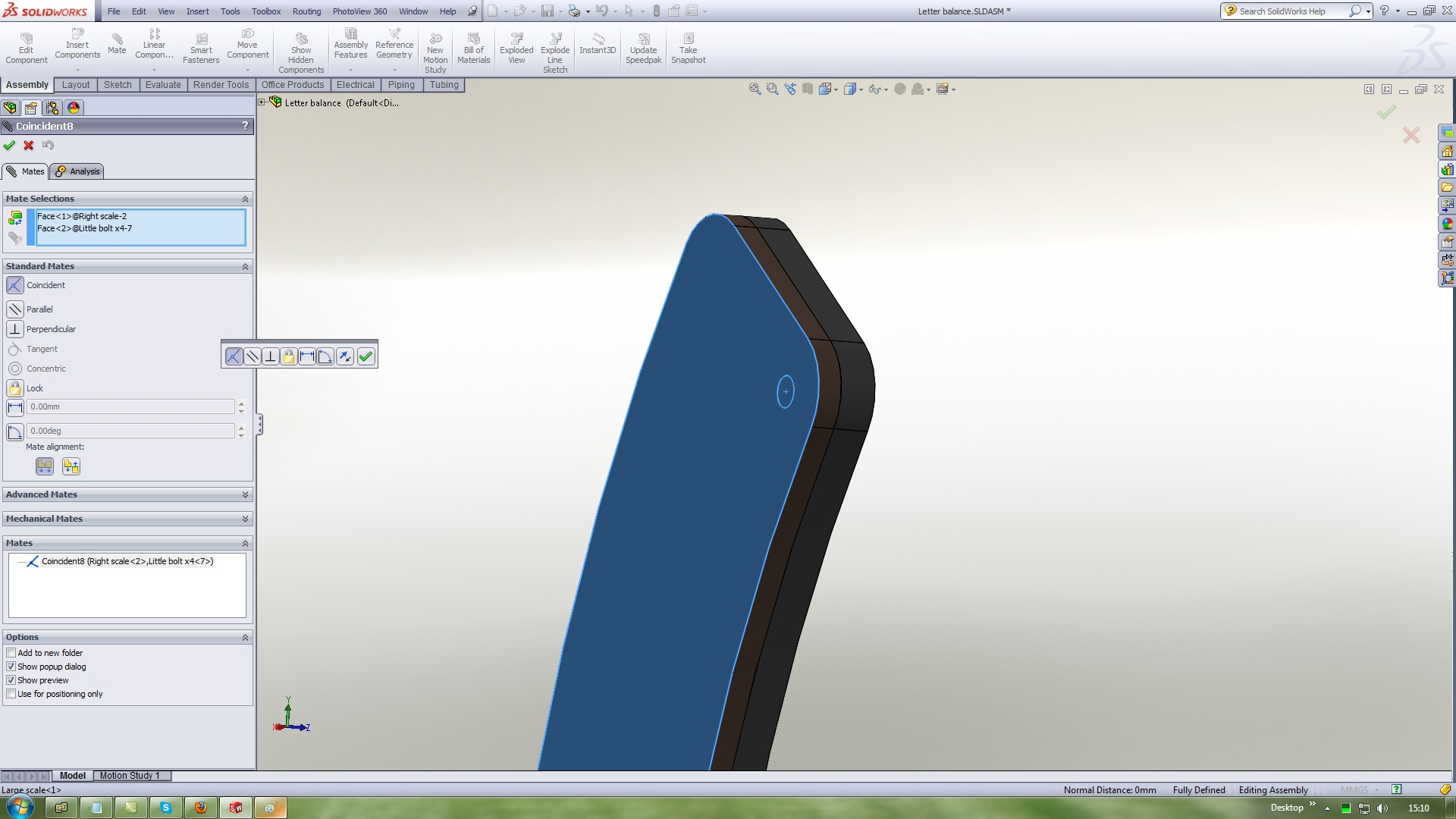8. ### Step 8:

Parallel mate this twoo planes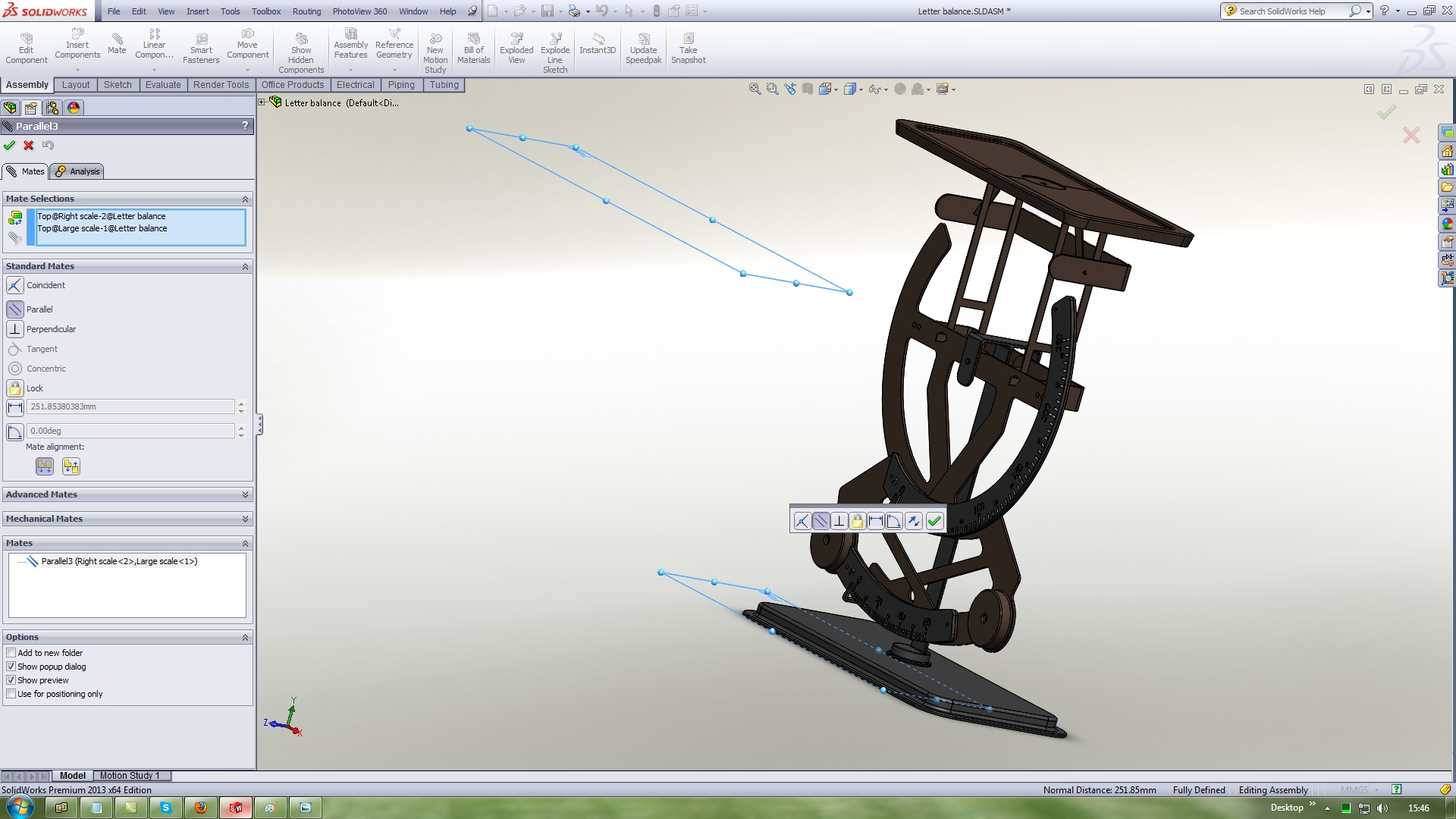9. ### Step 9:

Coincident mate this twoo planes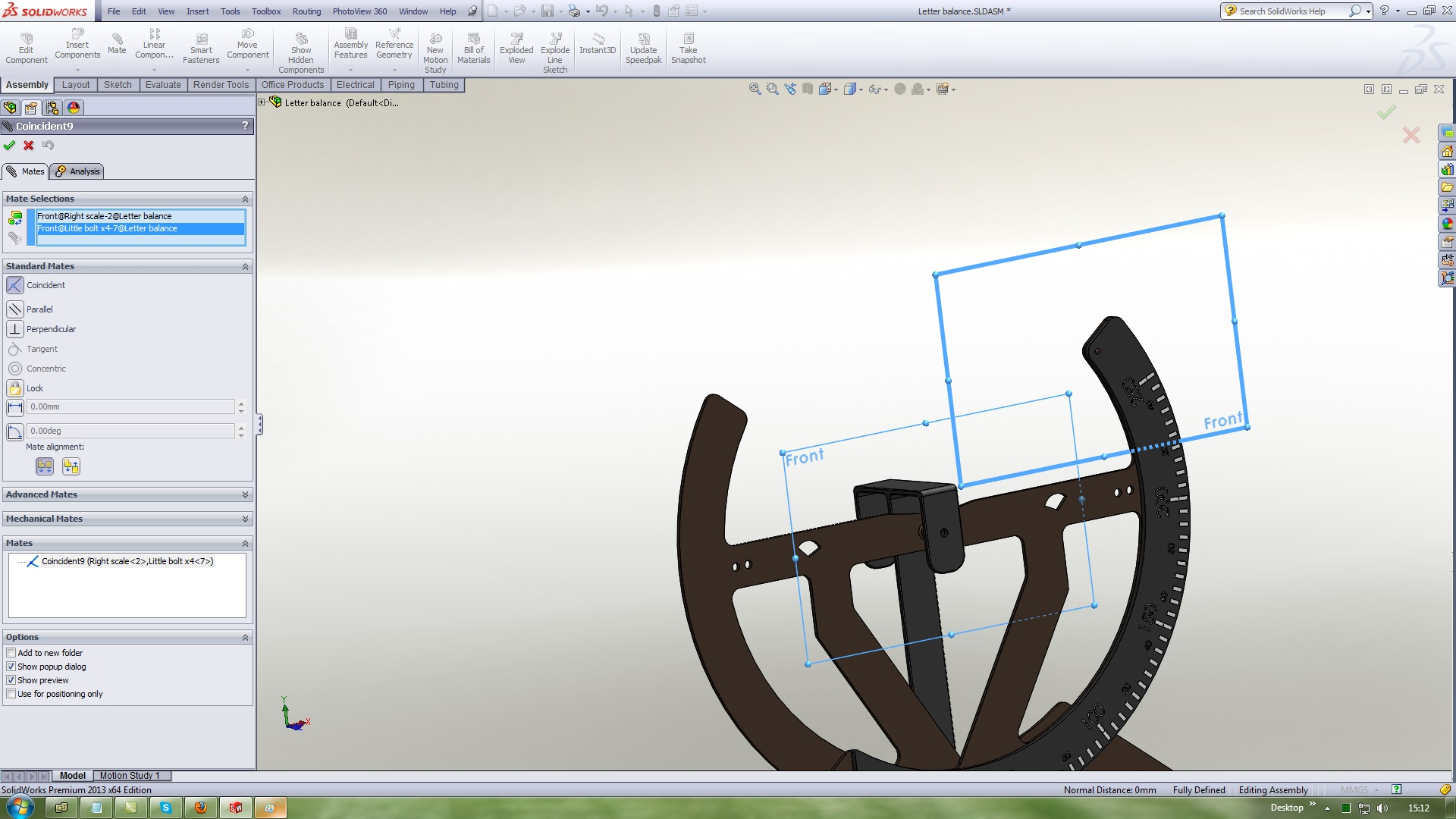10. ### Step 10:

Parallel mate this twoo planes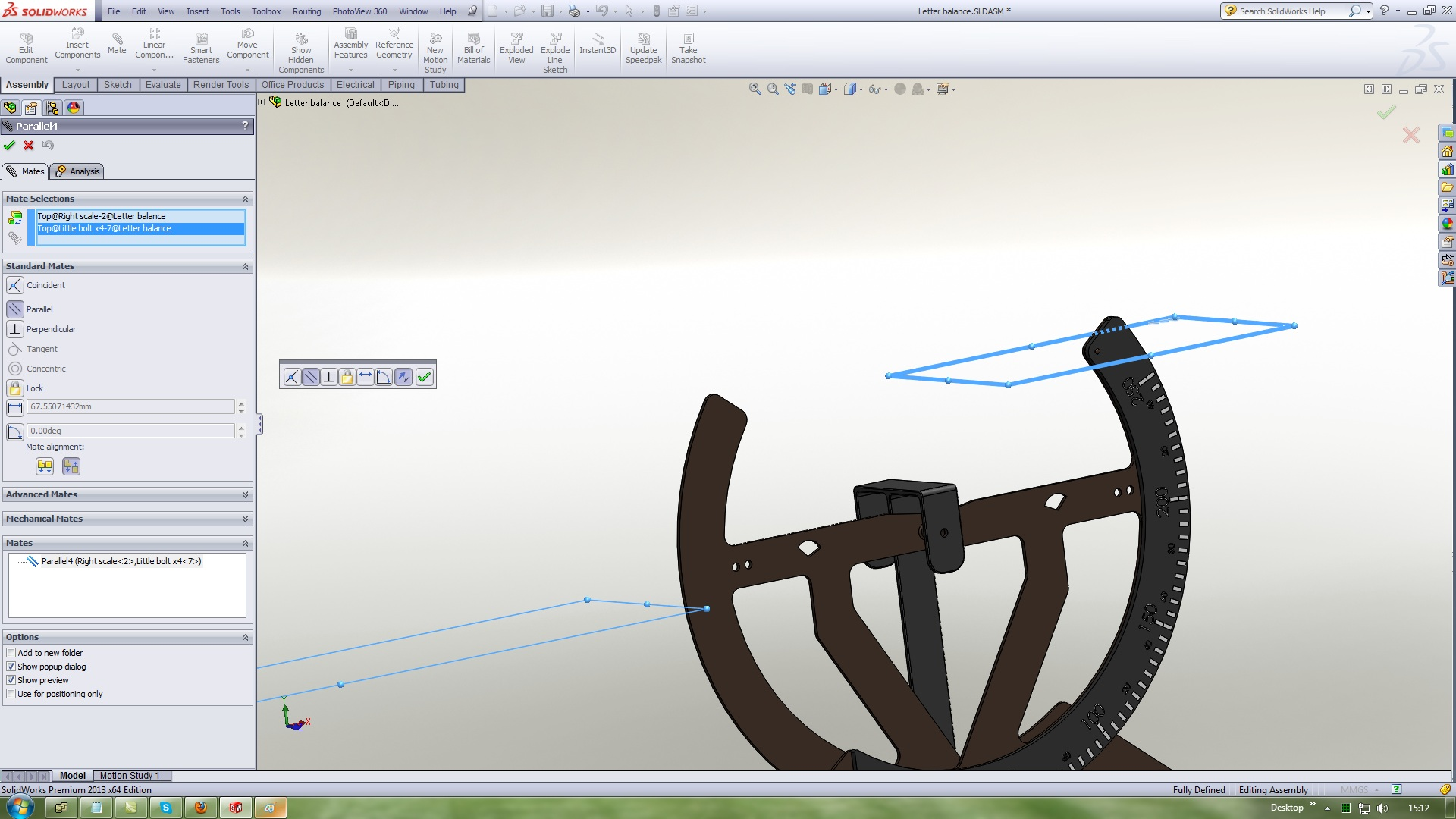11. ### Step 11:

Insert a Little bolt 2
Use Standard mates Concentric with this references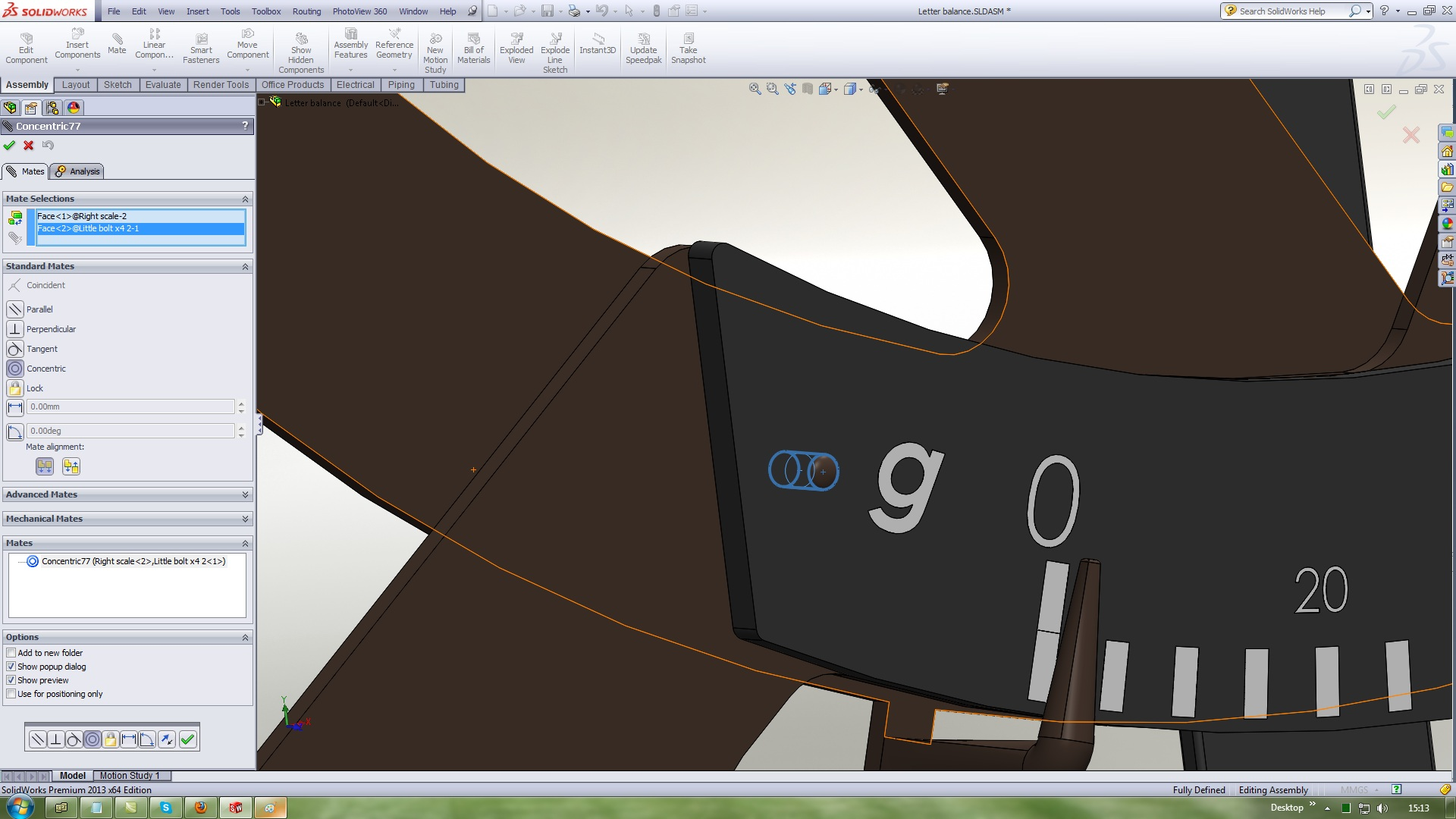12. ### Step 12:

Use Standard mates Coincident with this references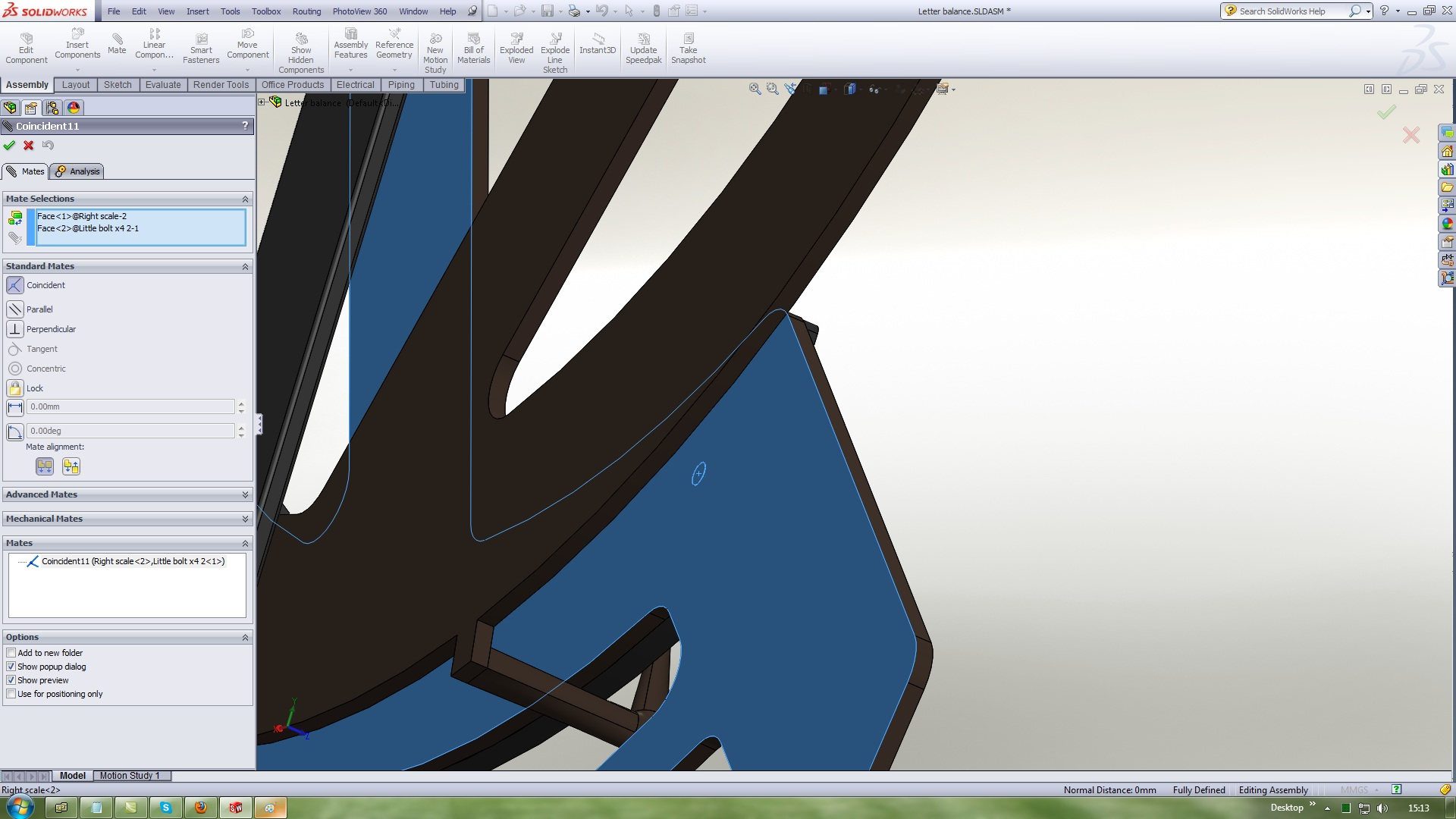13. ### Step 13:

Parallel mate this twoo planes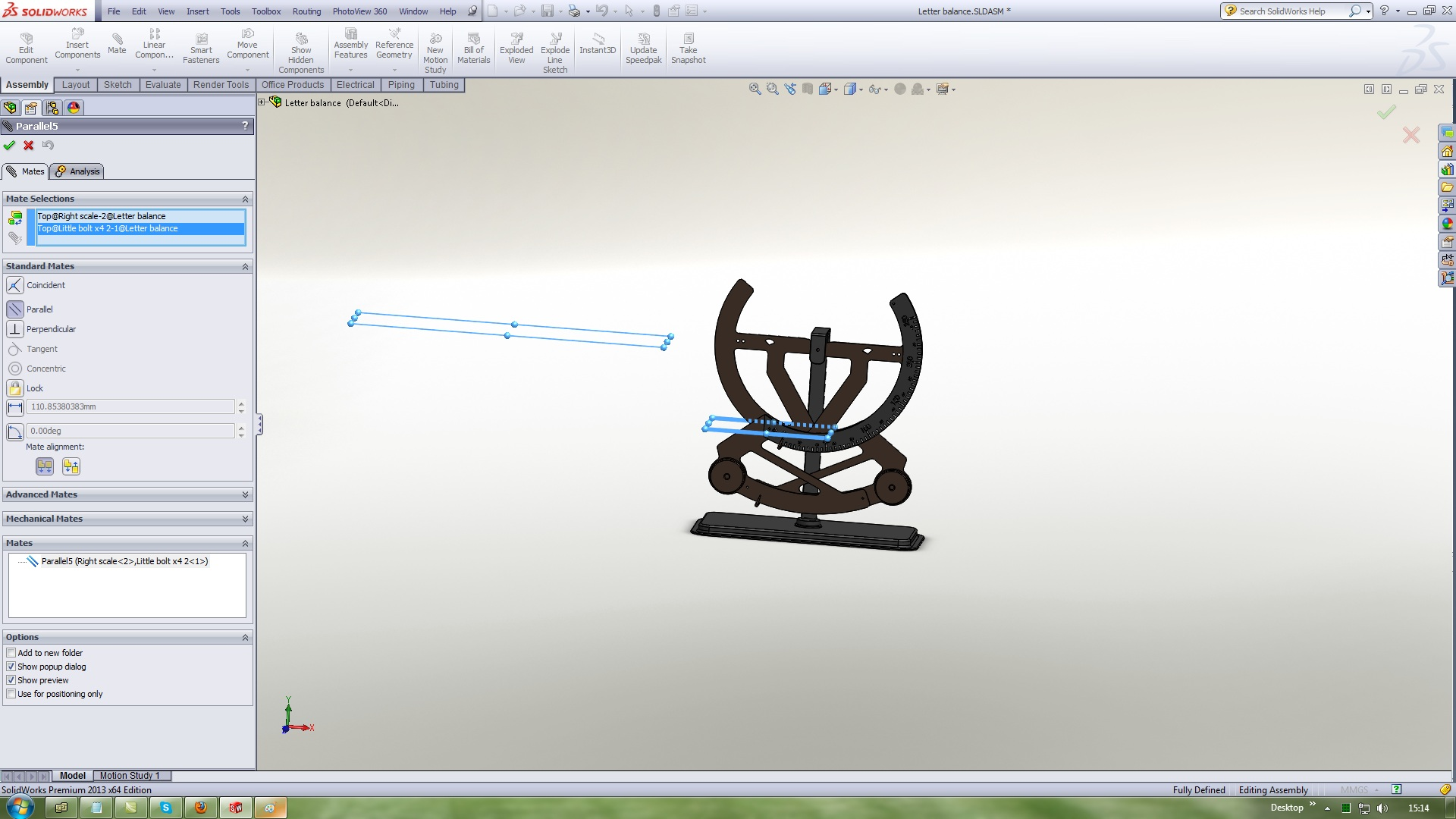14. ### Step 14:

Insert Little scale
Use Standard mates Concentric with this references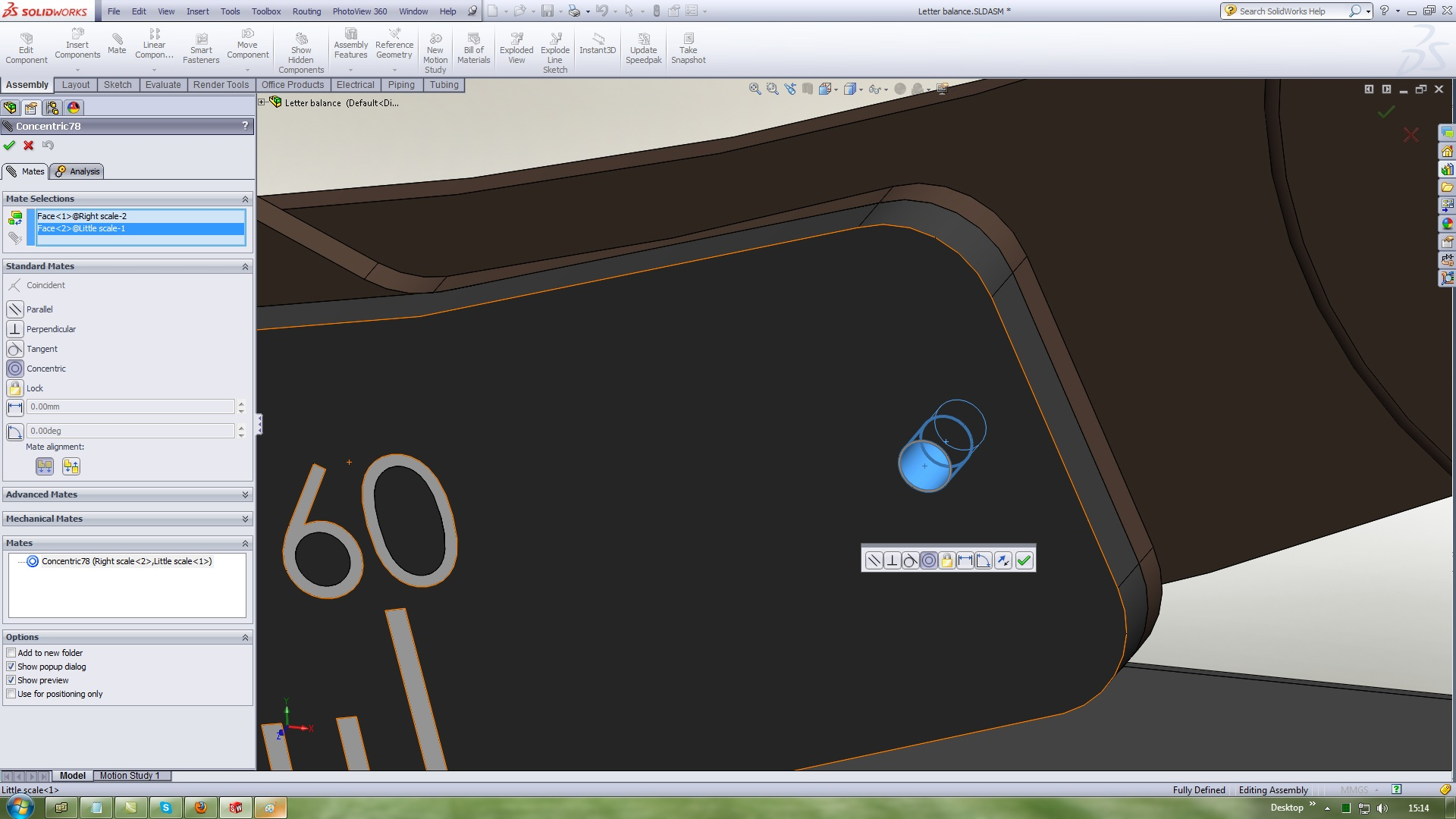15. ### Step 15:

Other holle the same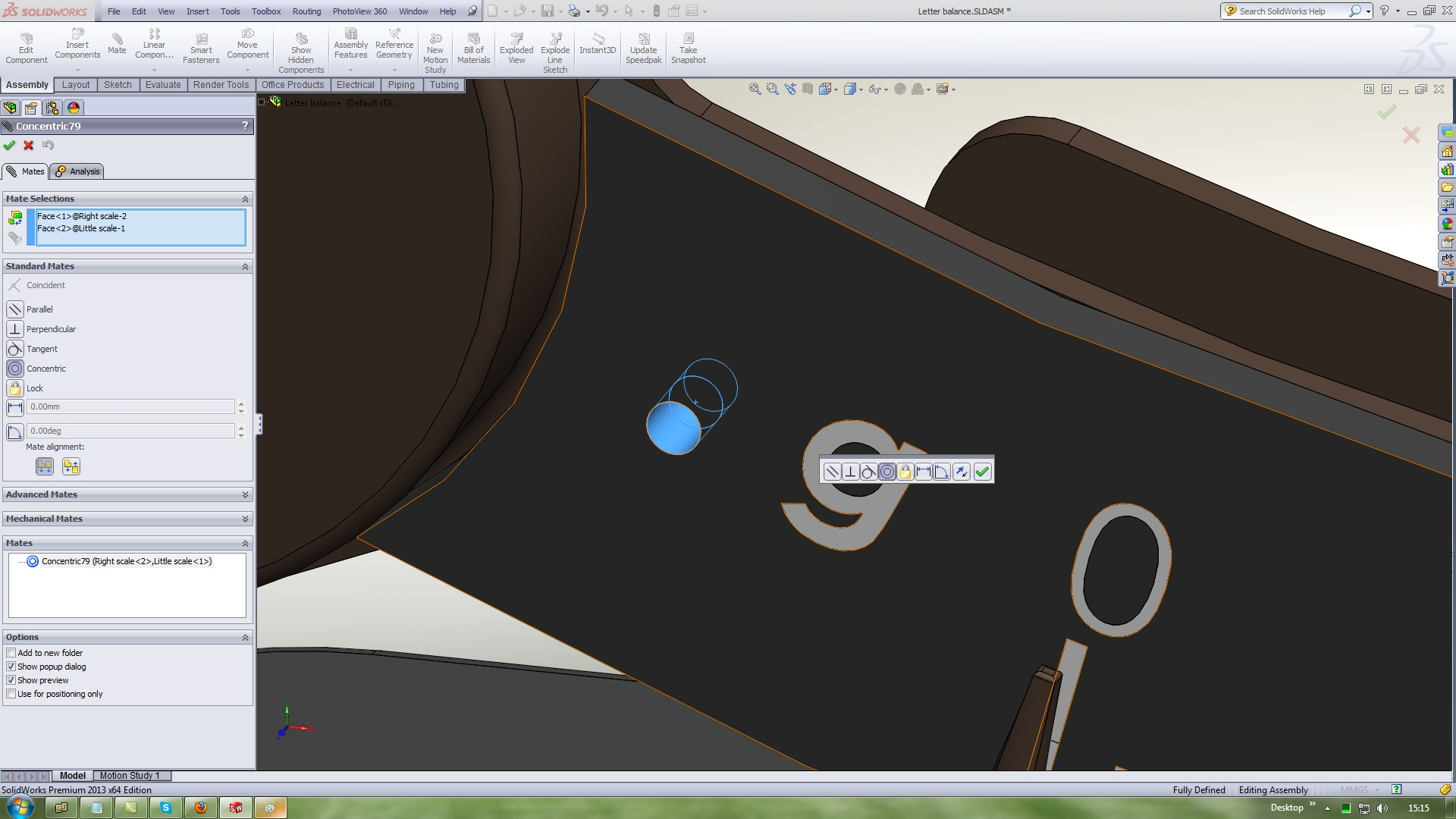16. ### Step 16:

Use Standard mates Coincident with this references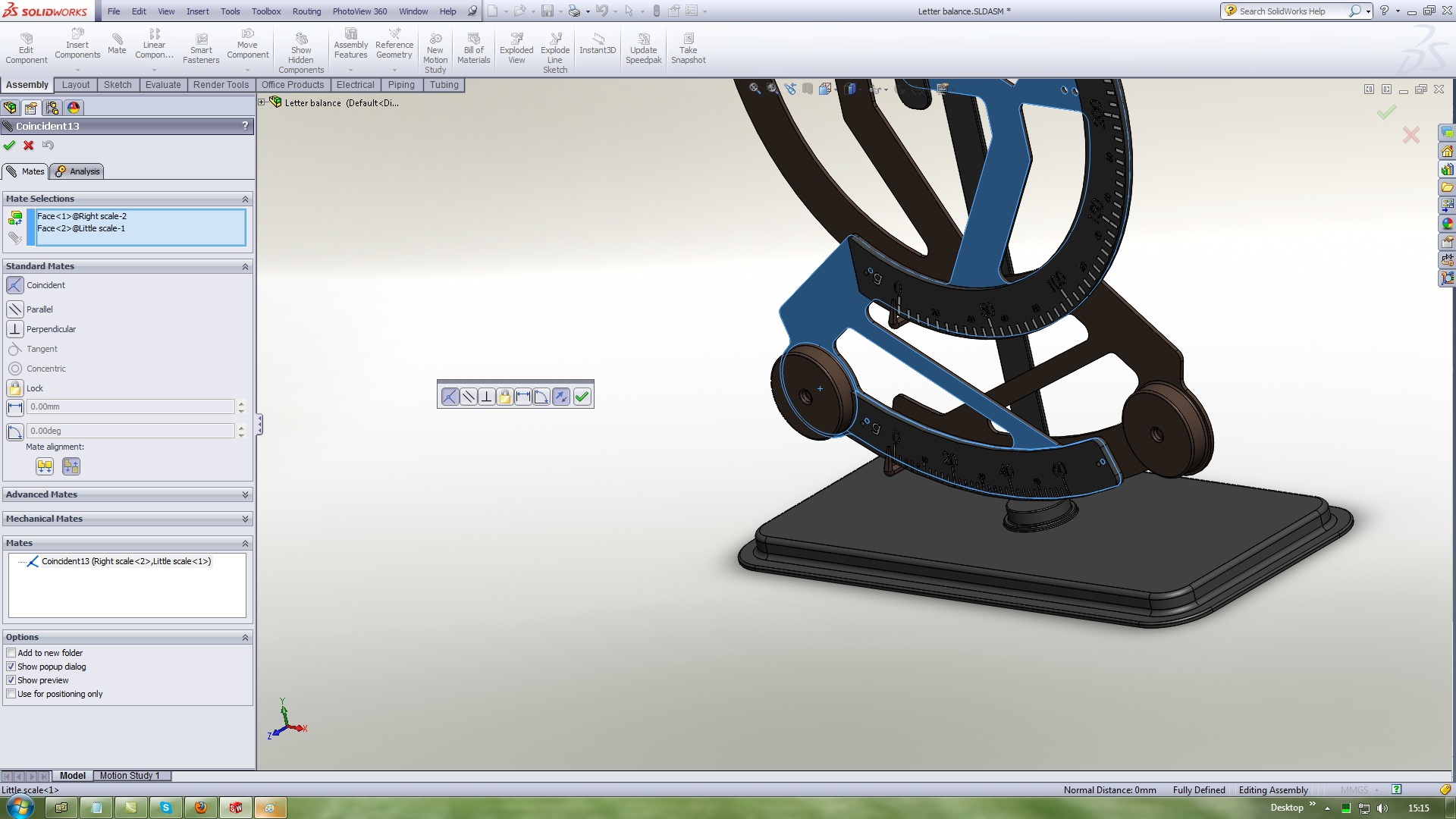17. ### Step 17:

Insert a Little bolt 3
Use Standard mates Concentric with this references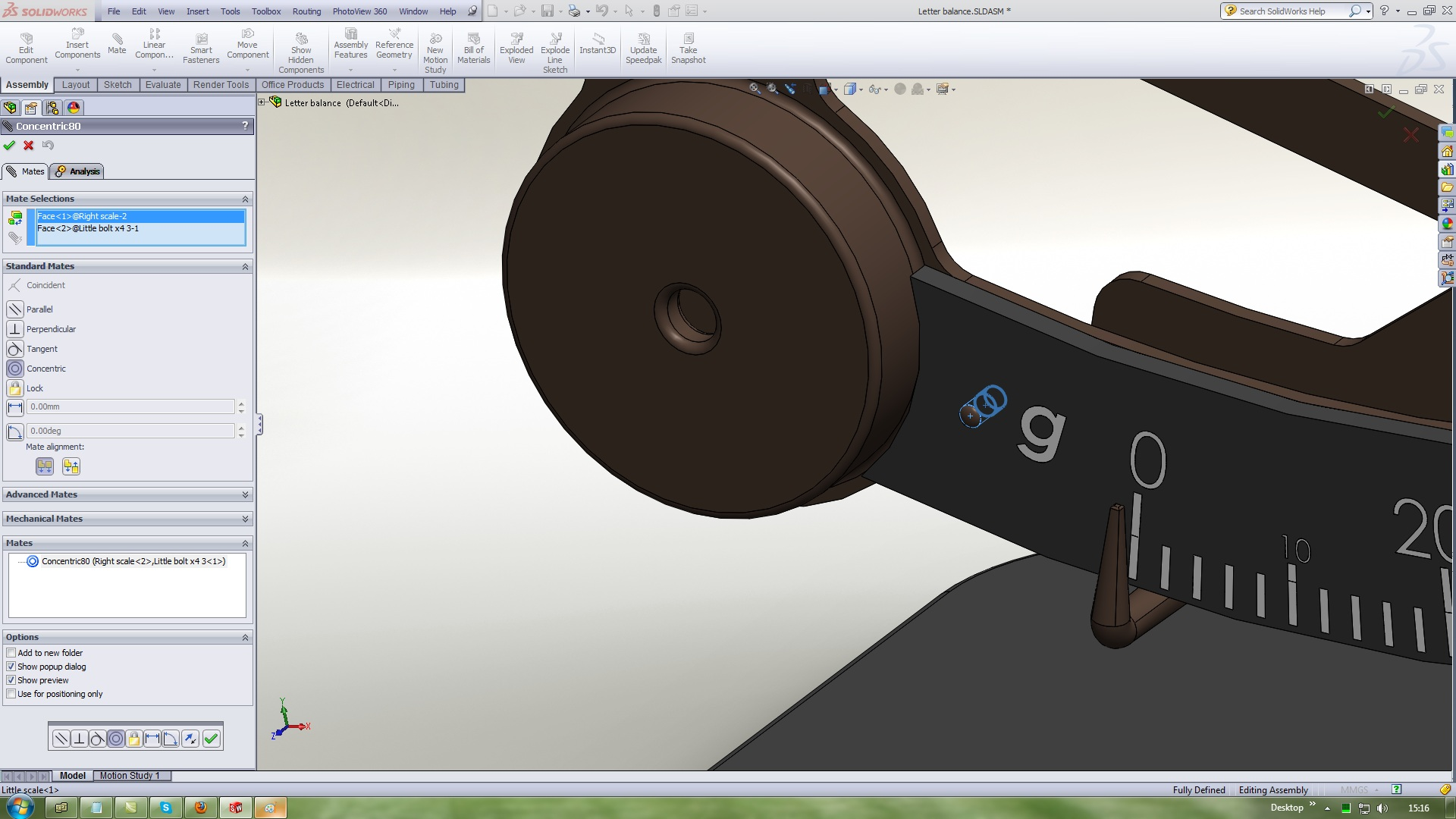18. ### Step 18:

Use Standard mates Coincident with this references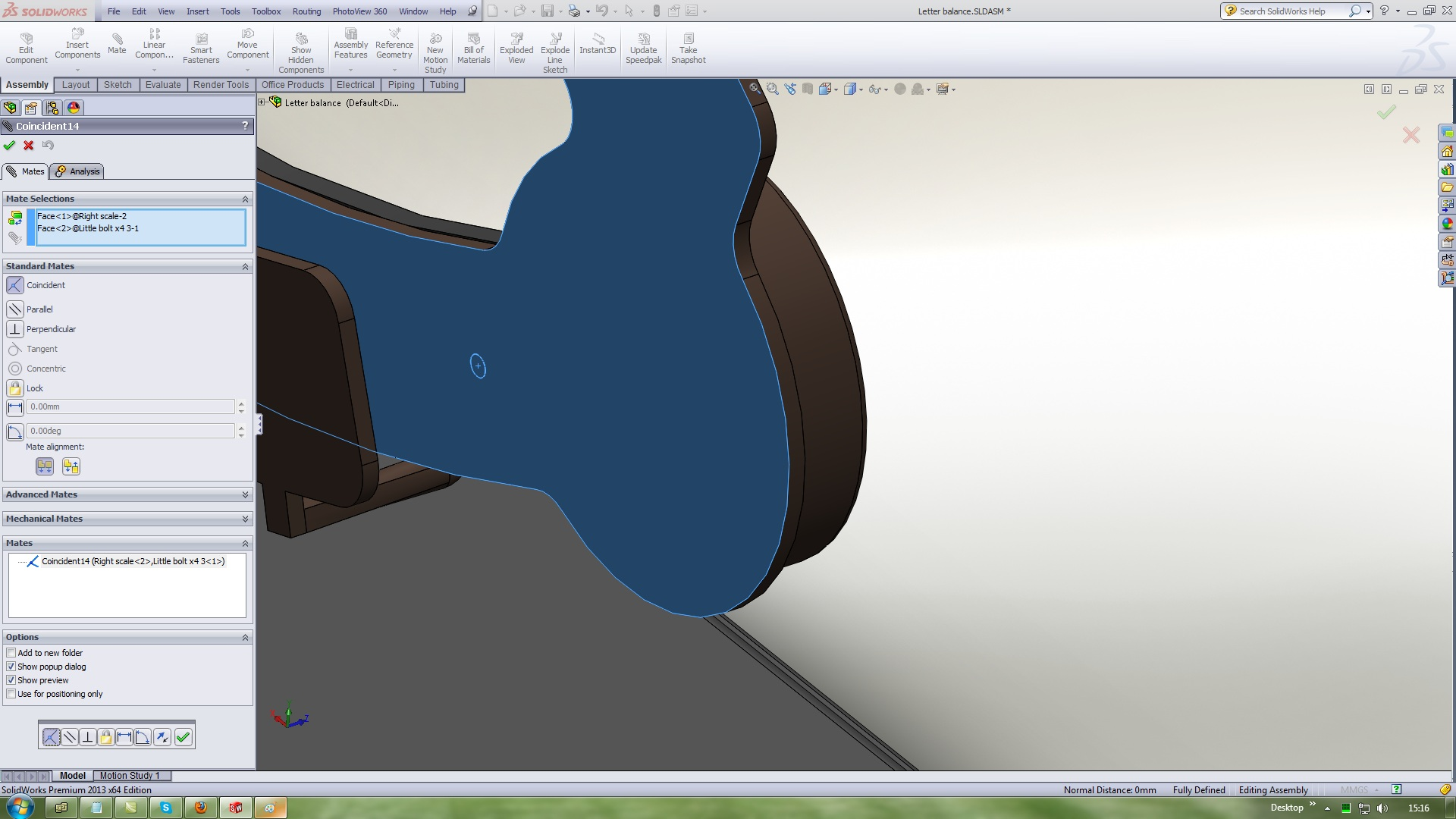19. ### Step 19:

Parallel mate this twoo planes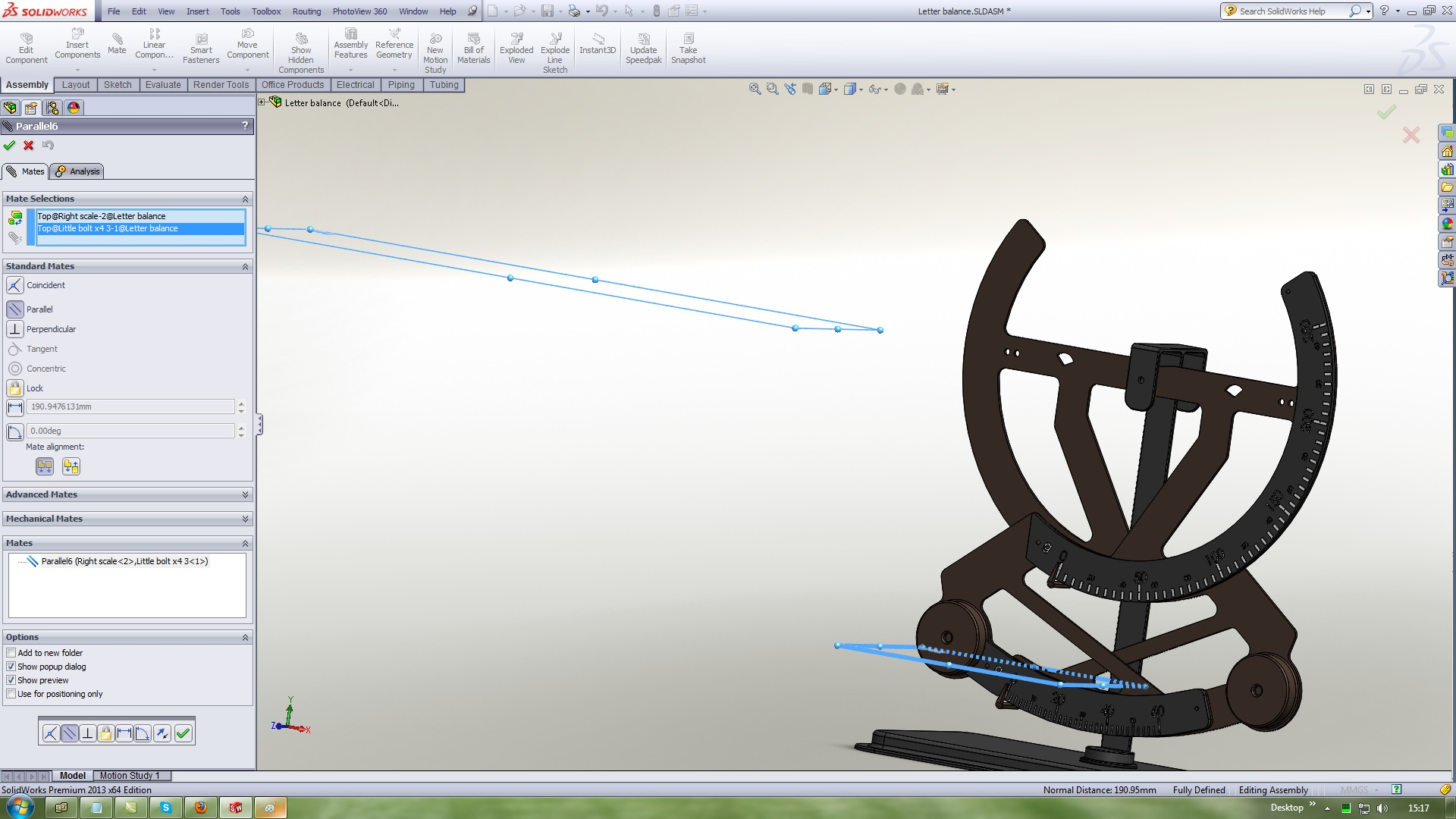20. ### Step 20:

Insert a Little bolt 4
Use Standard mates Concentric with this references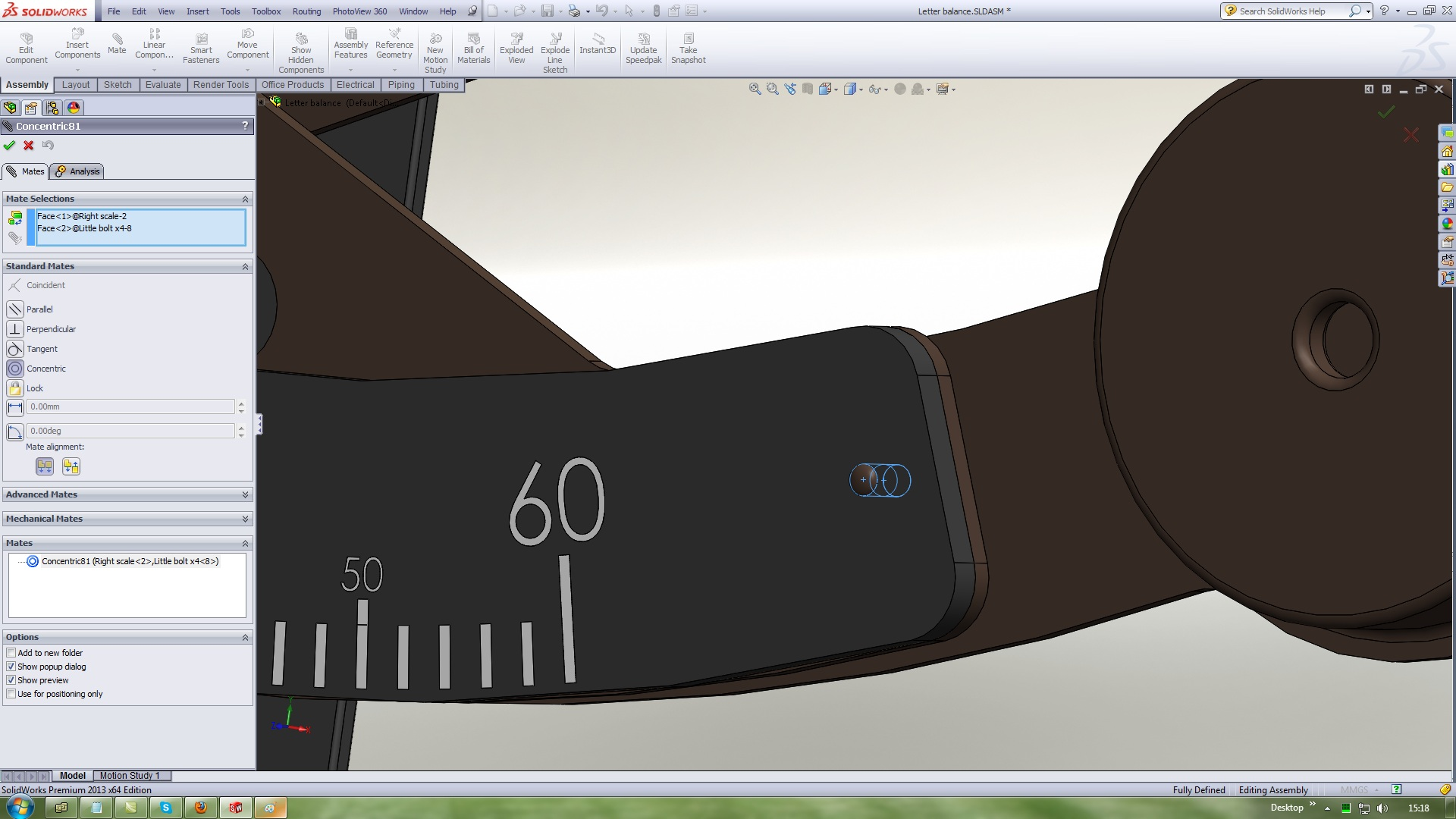21. ### Step 21:

Use Standard mates Coincident with this references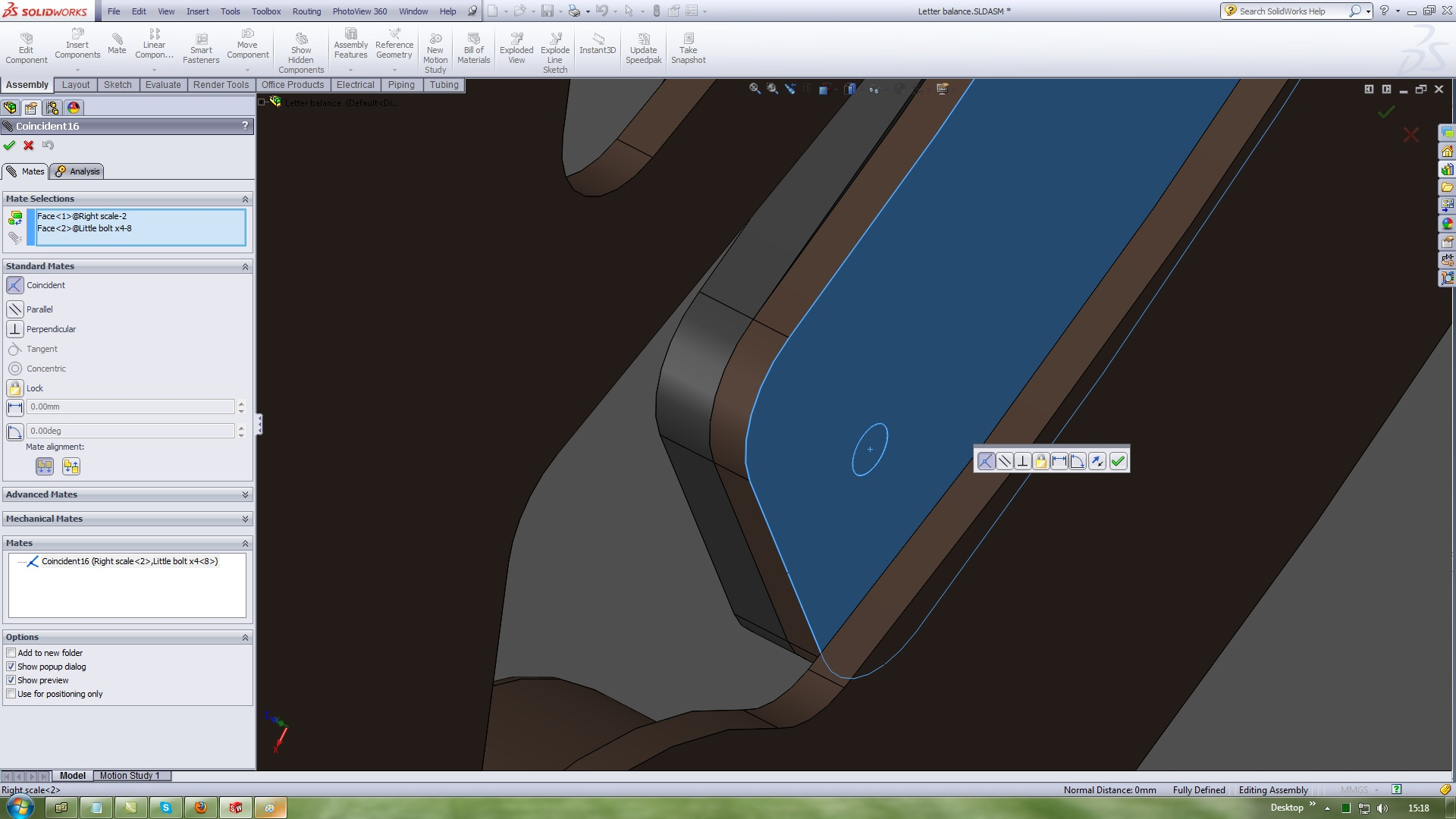22. ### Step 22:

Parallel mate this twoo planes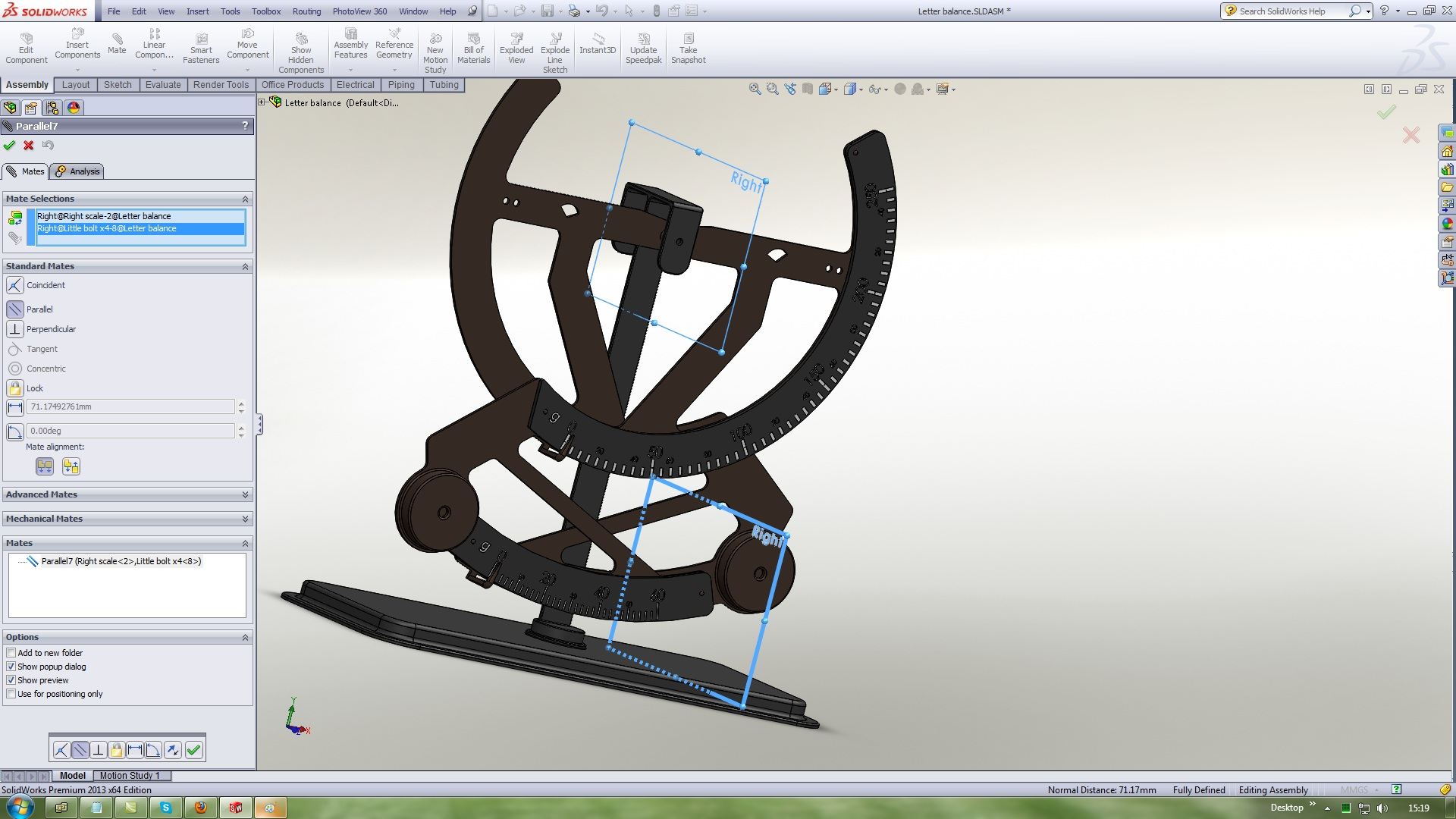23. ### Step 23:

Insert a Left hinge
Use Standard mates Concentric with this references24. ### Step 24:

Other holle the same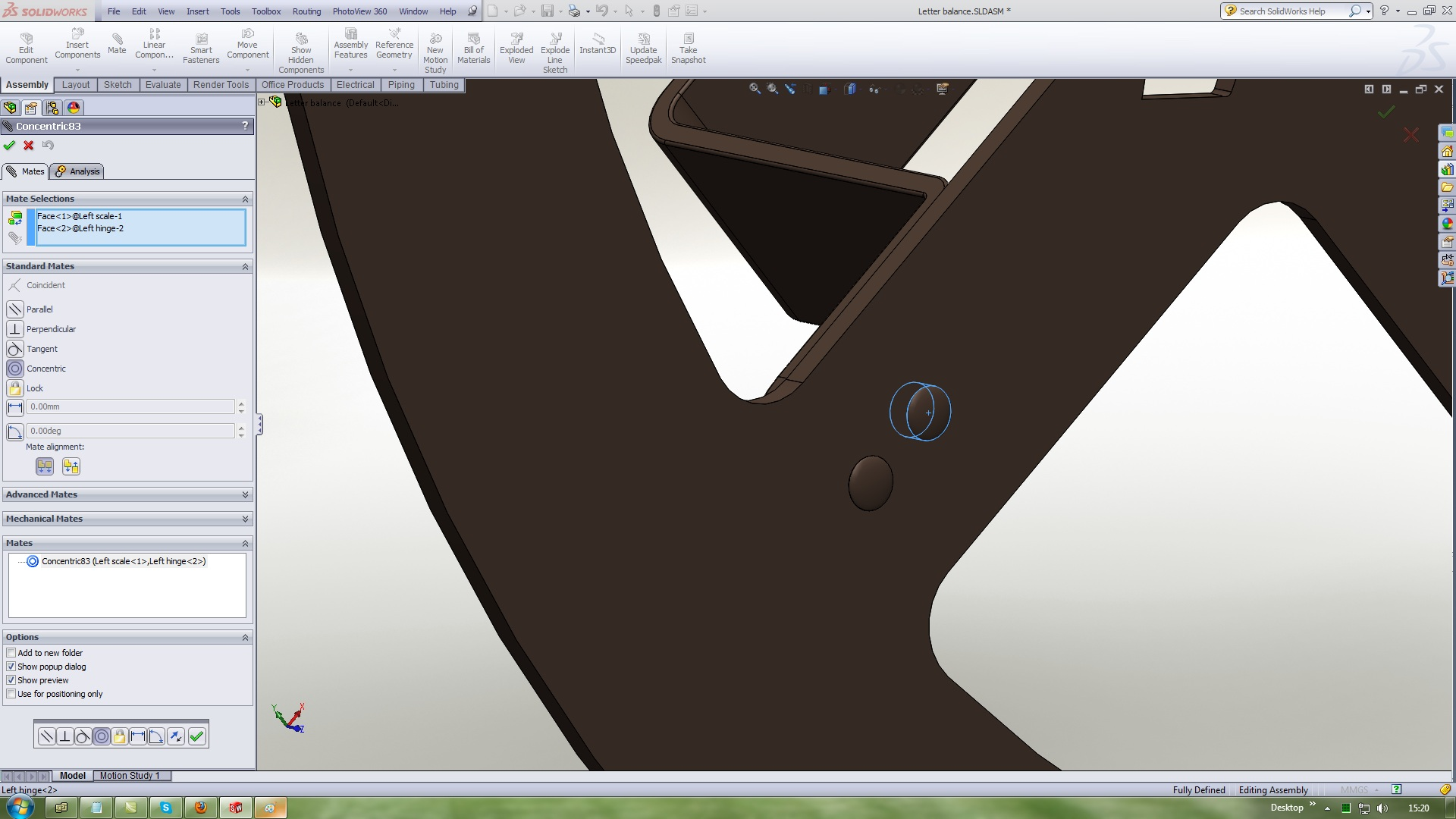25. ### Step 25:

Use Standard mates Coincident with this references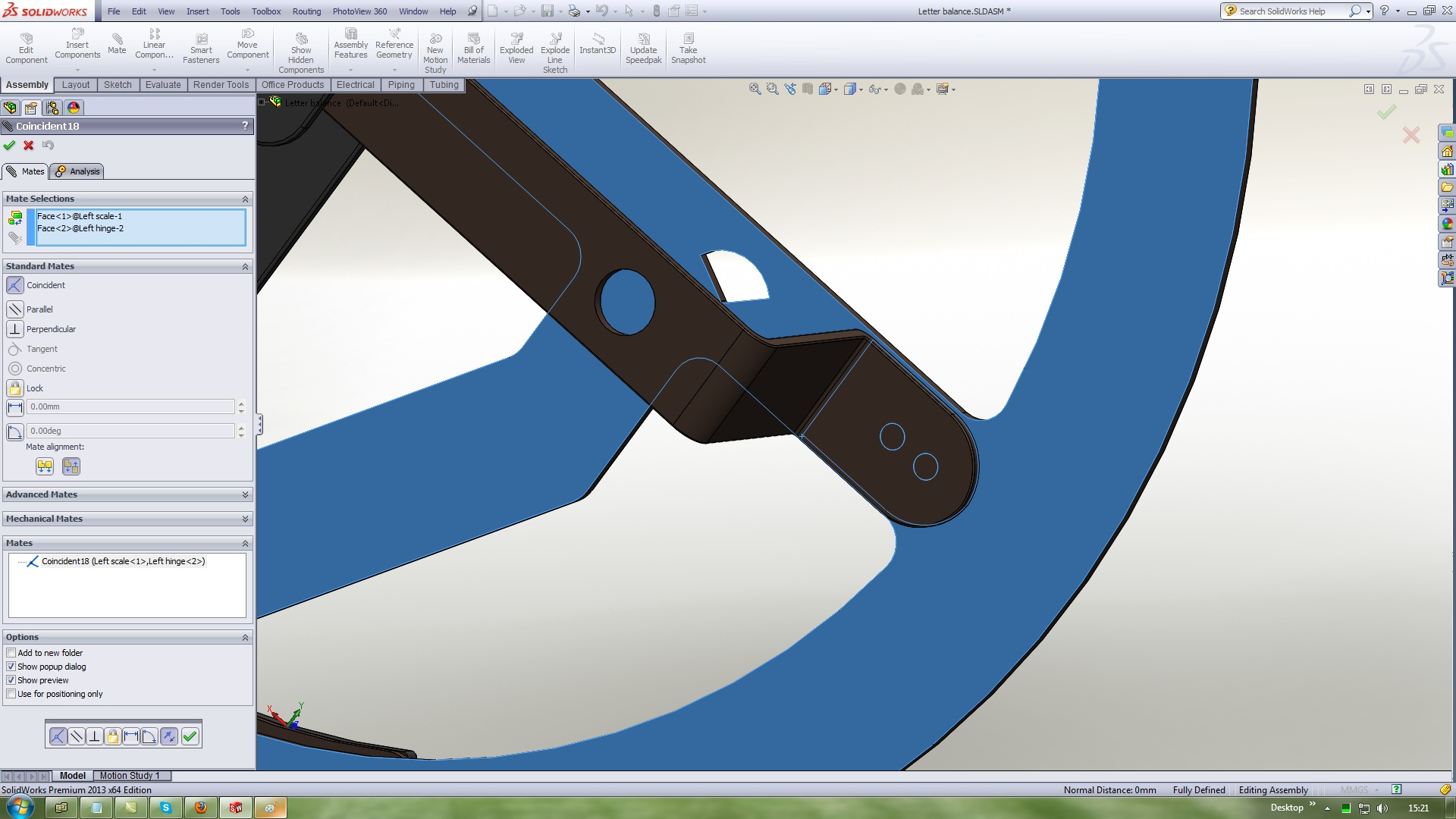26. ### Step 26:

Insert a Right hinge
Use Standard mates Concentric with this references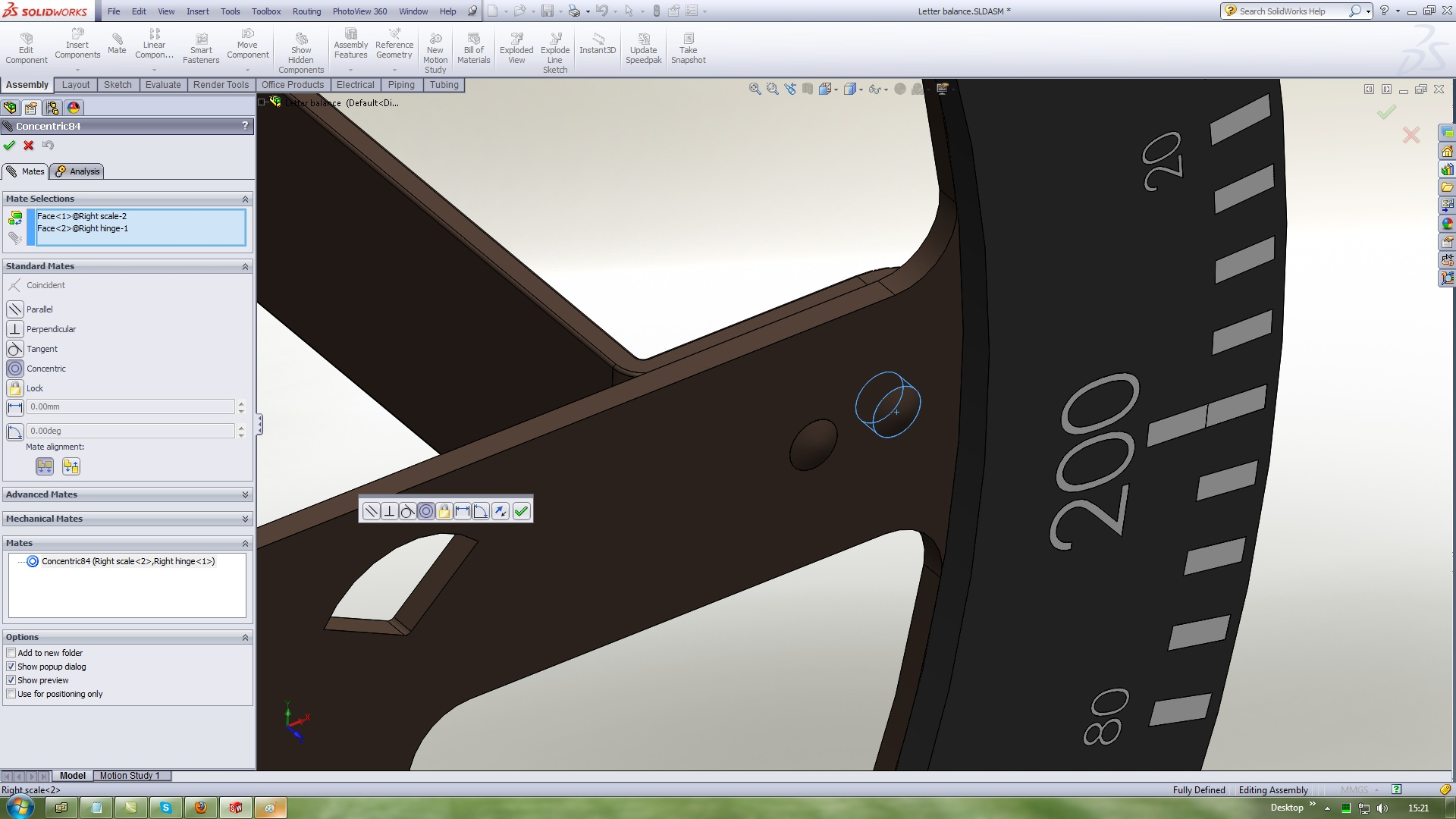27. ### Step 27:

Other holle the same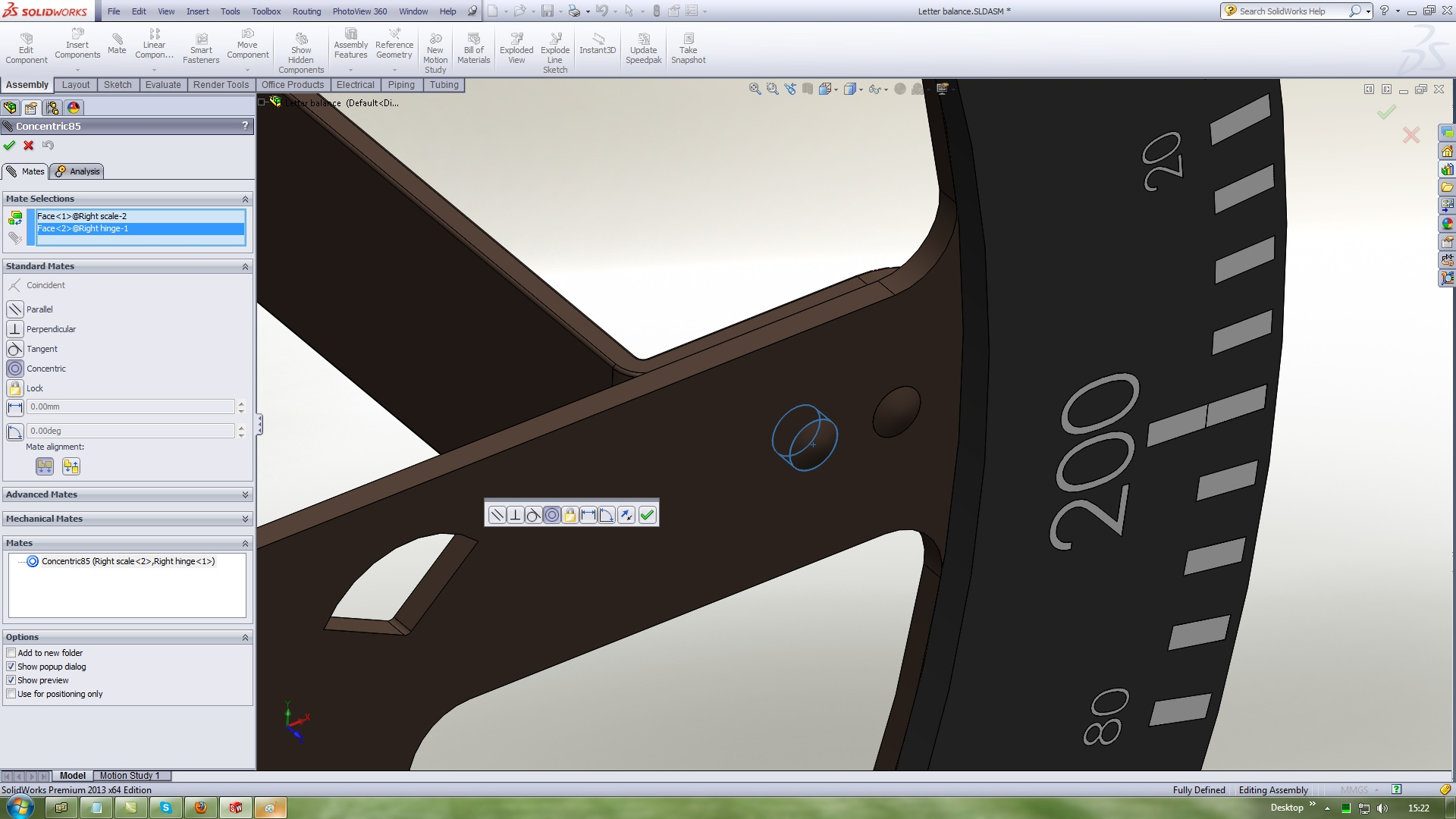28. ### Step 28:

Use Standard mates Coincident with this references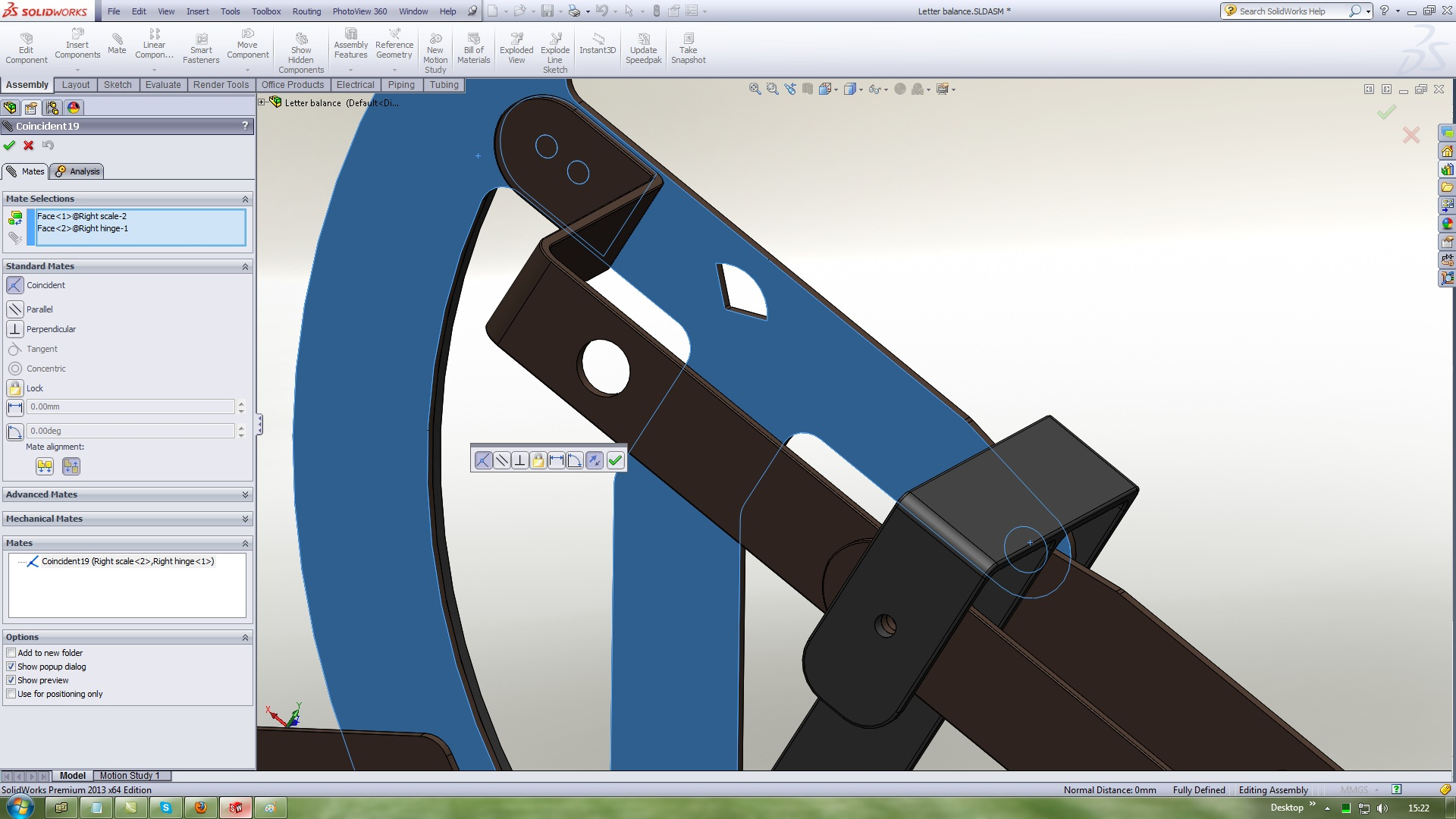29. ### Step 29:

Parallel mate this twoo planes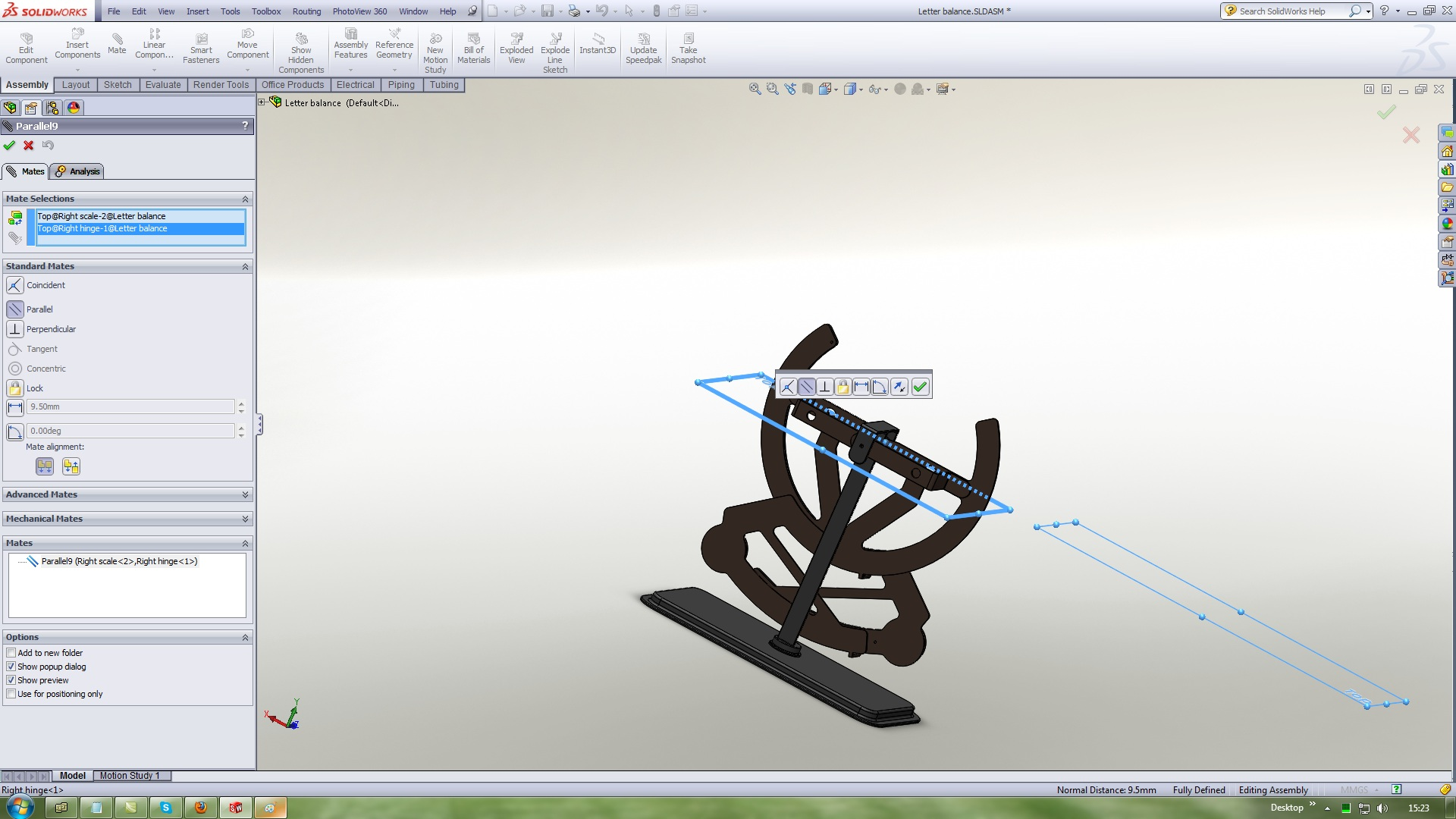30. ### Step 30:

Insert a Bolt
Use Standard mates Concentric with this references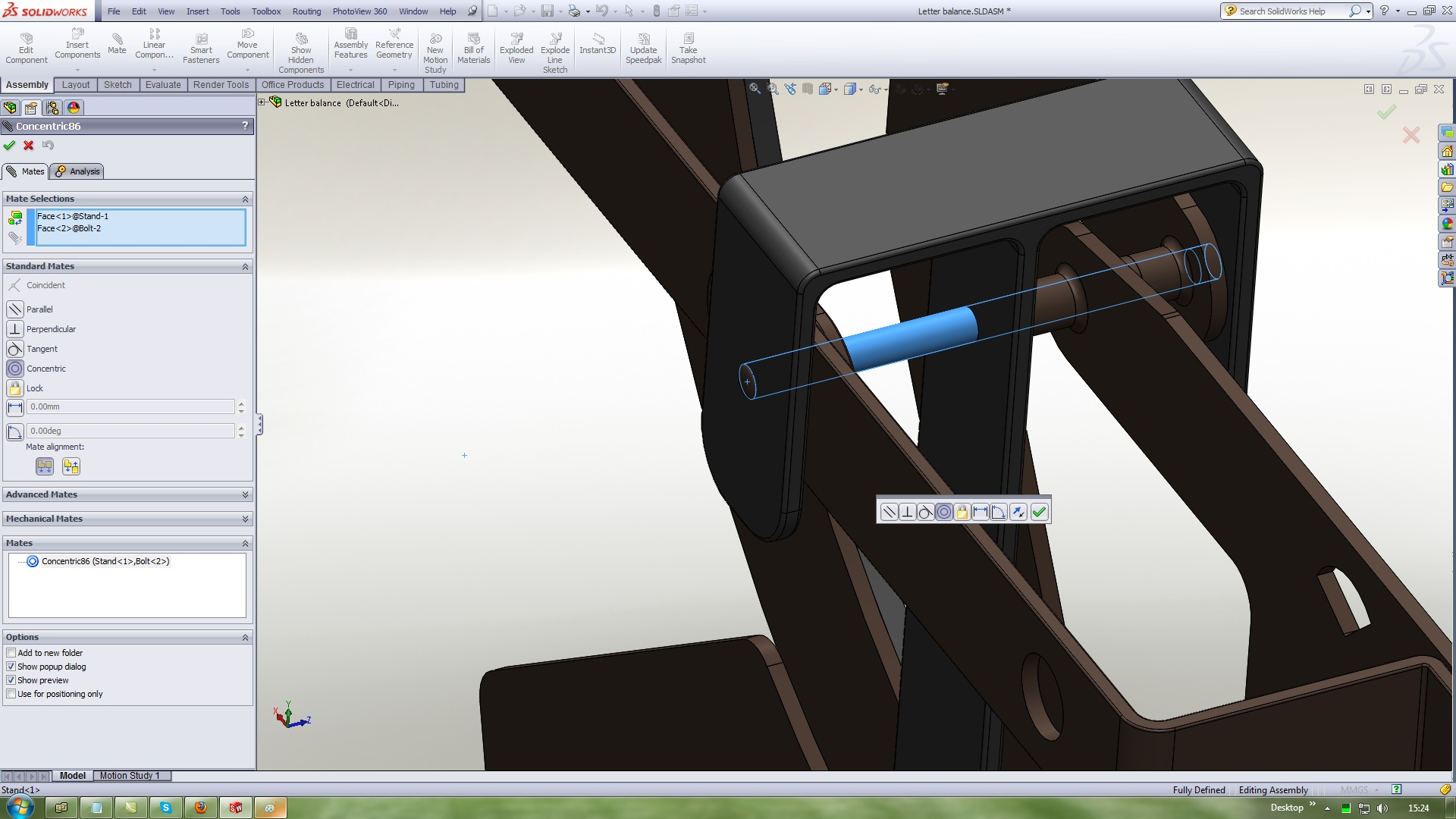31. ### Step 31:

Use Standard mates Coincident with this references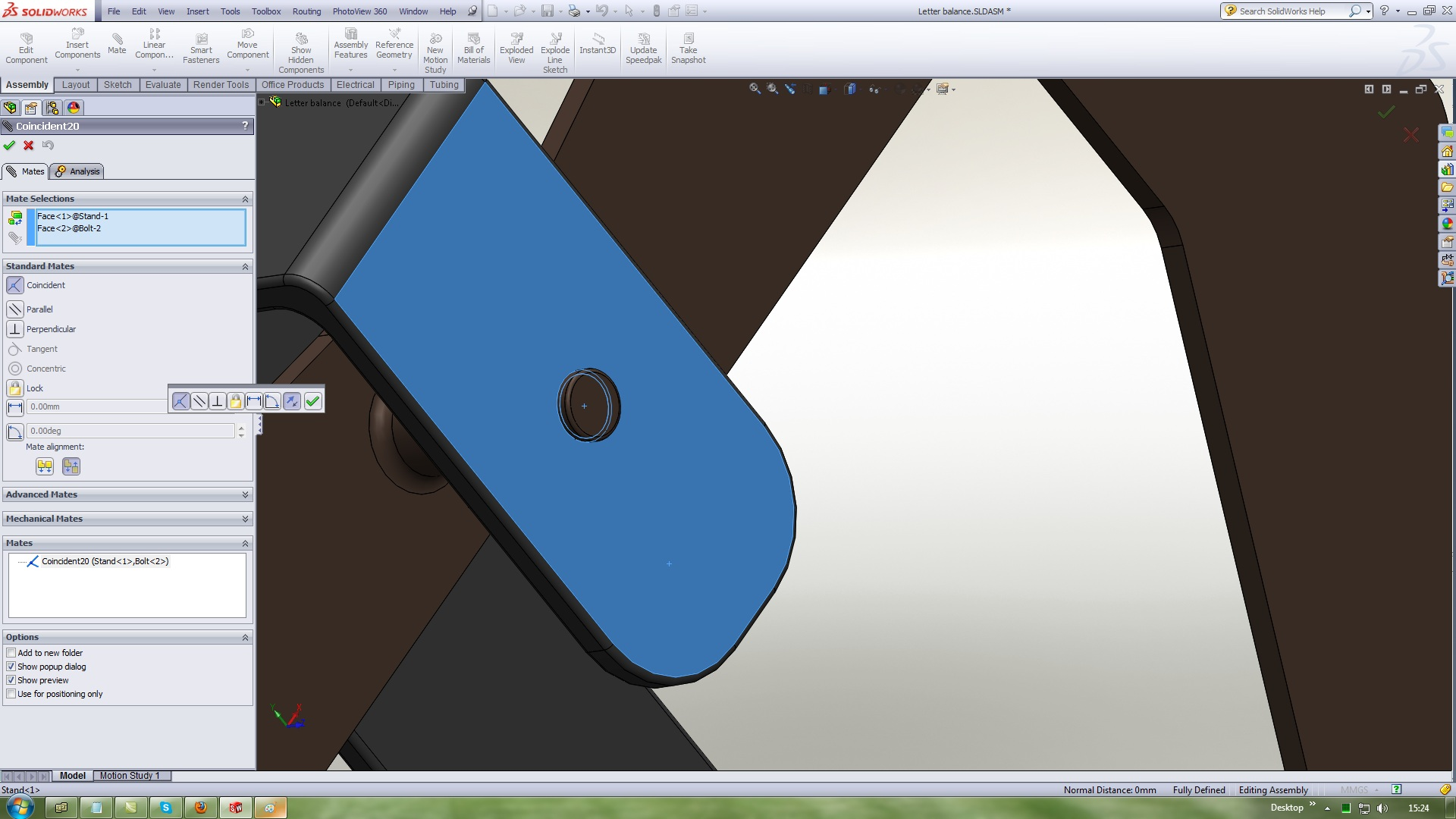32. ### Step 32:

Parallel mate this twoo planes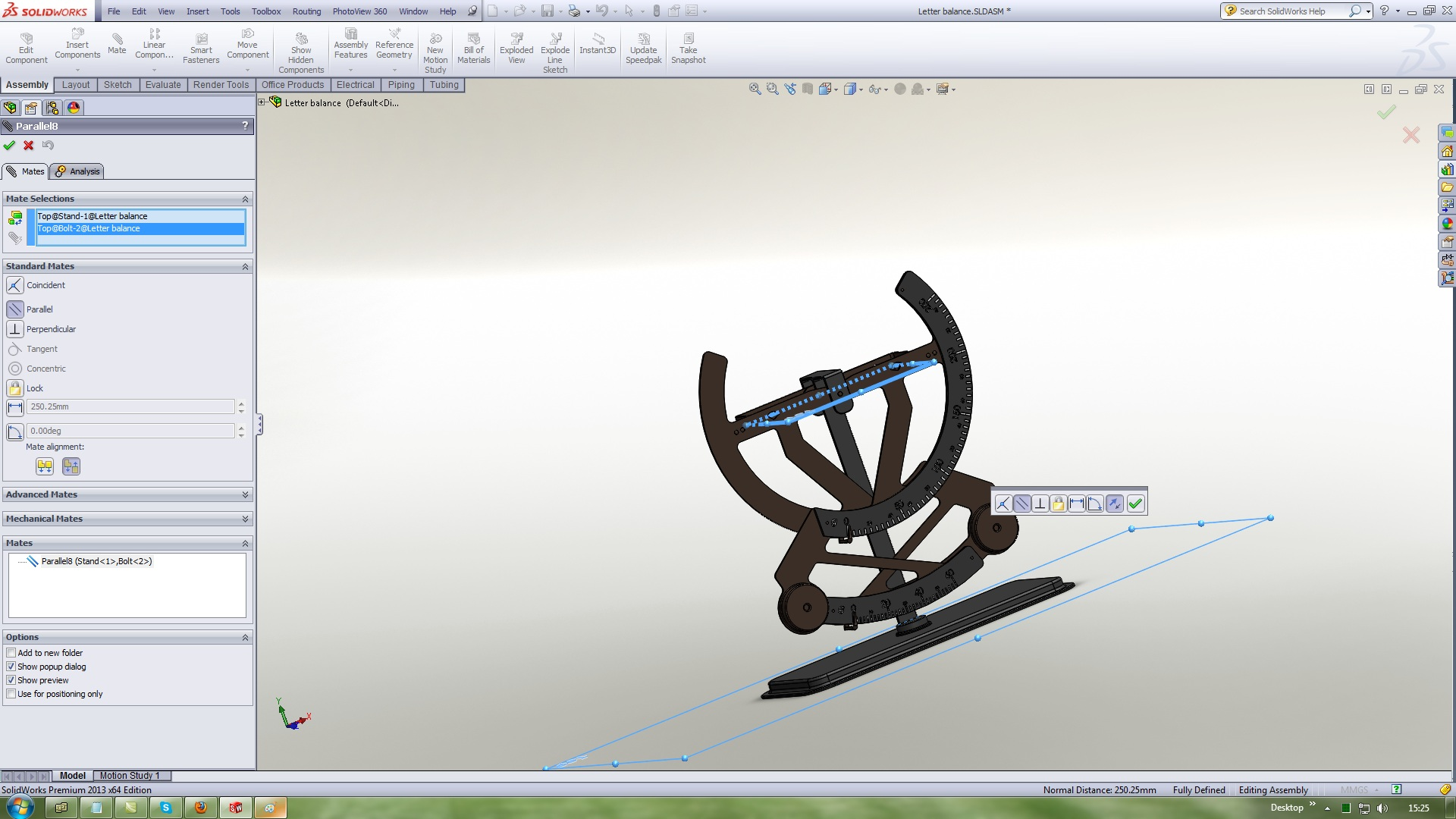33. ### Step 33:

Insert a Right crank
Use Standard mates Concentric with this references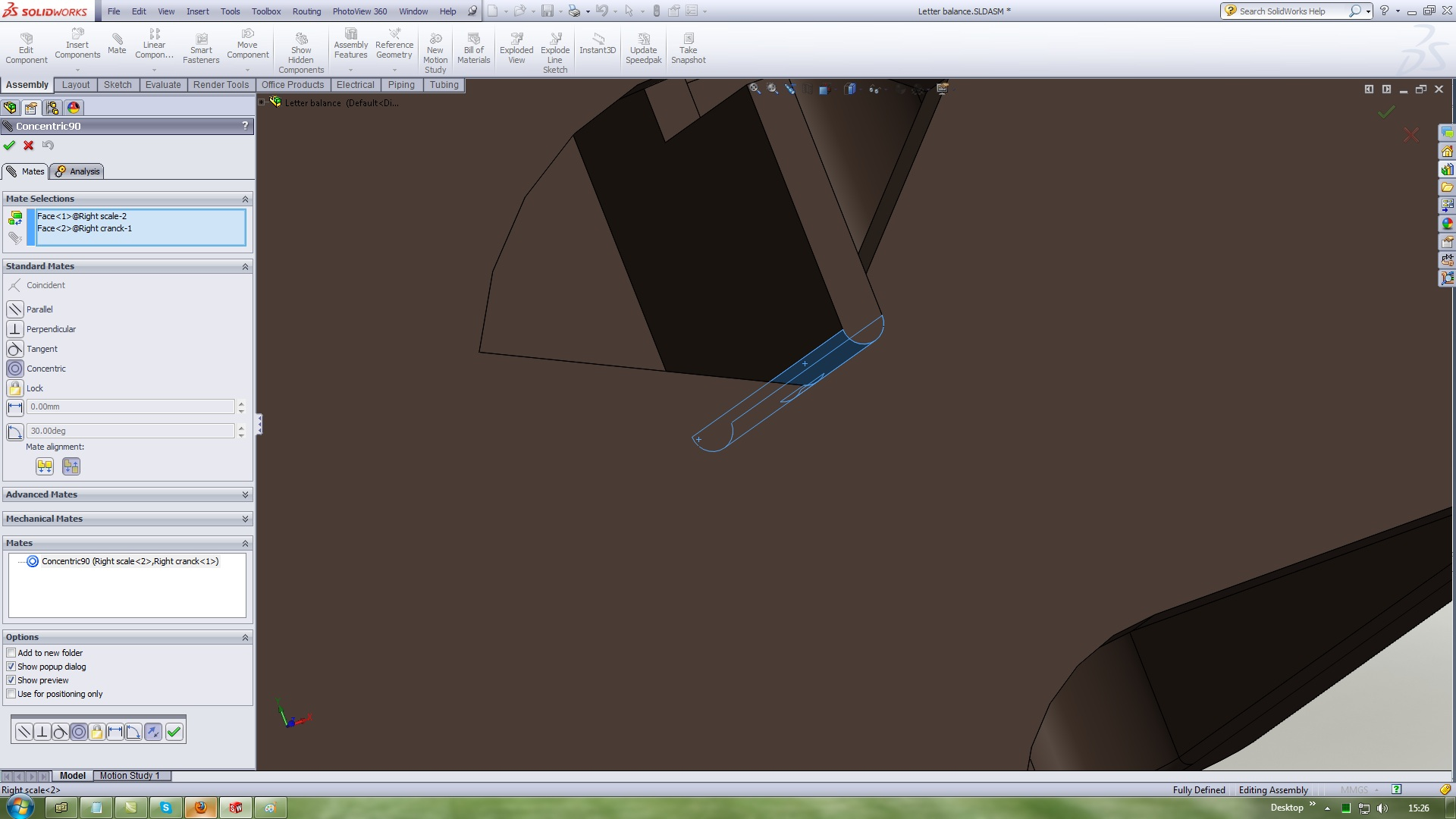34. ### Step 34:

Insert a Left crank
Use Standard mates Concentric with this references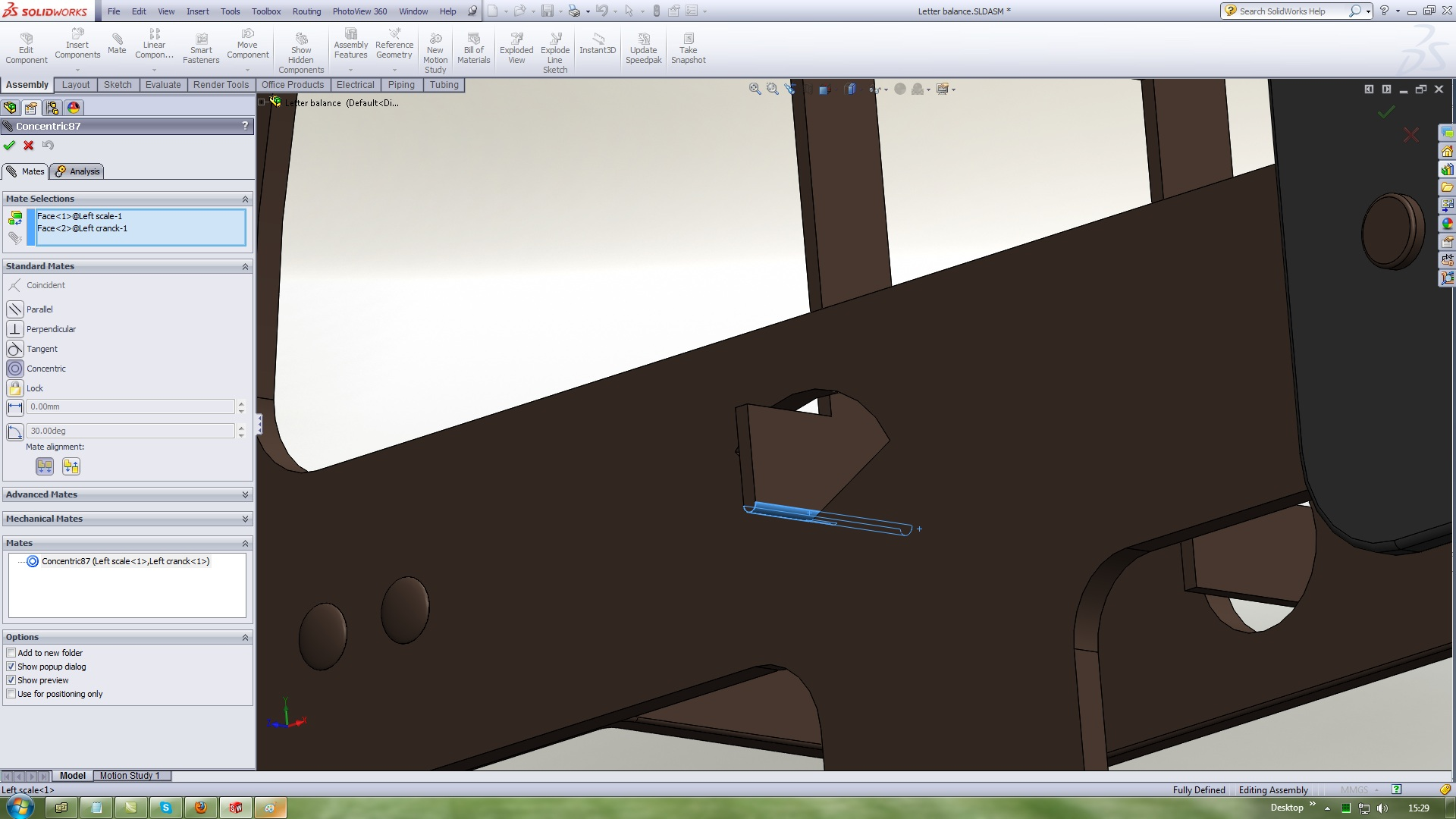35. ### Step 35:

Insert a Top
Use Standard mates Coincident and mate this twoo planes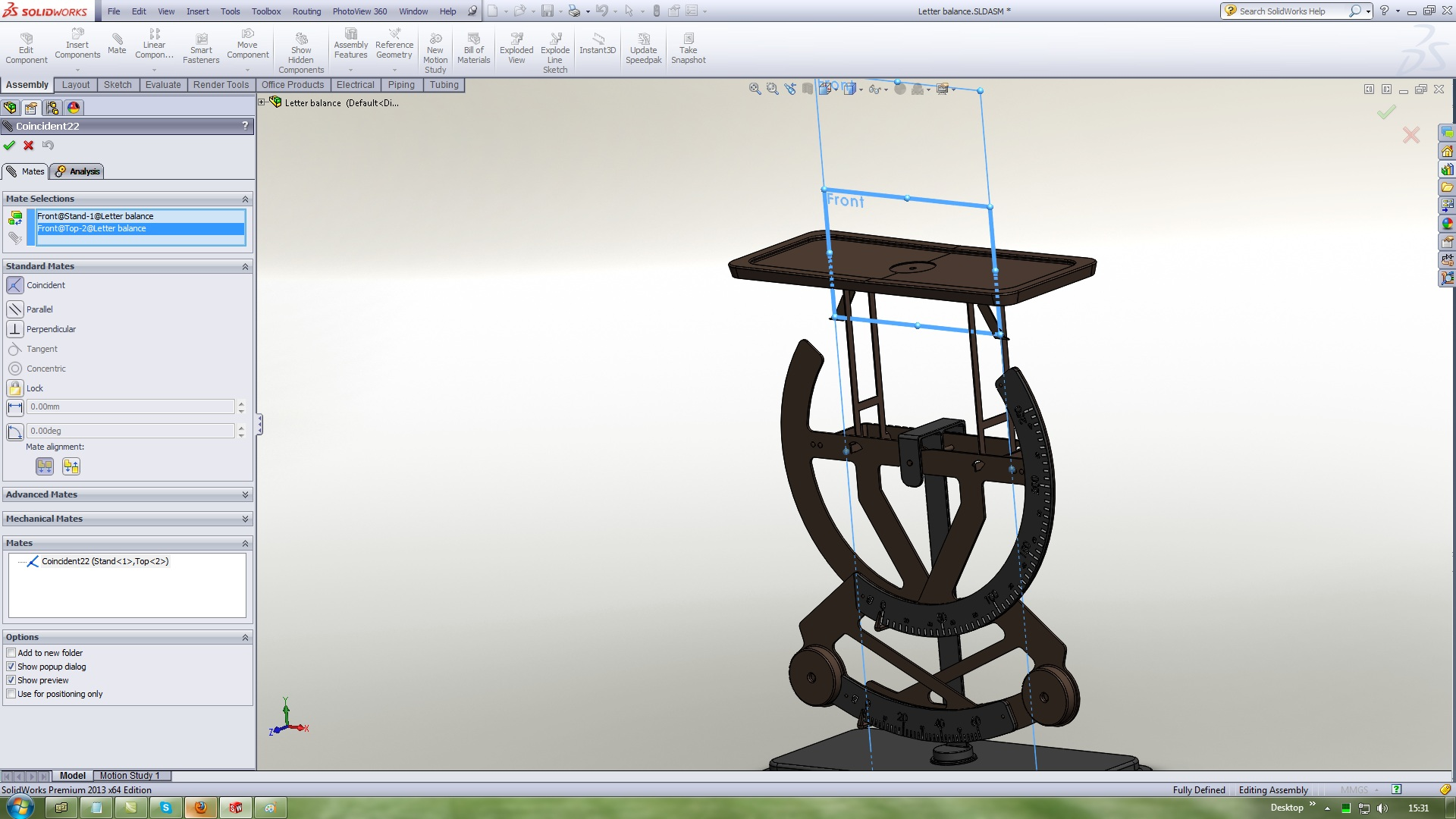36. ### Step 36:

Use Standard mates Coincident and mate this twoo planes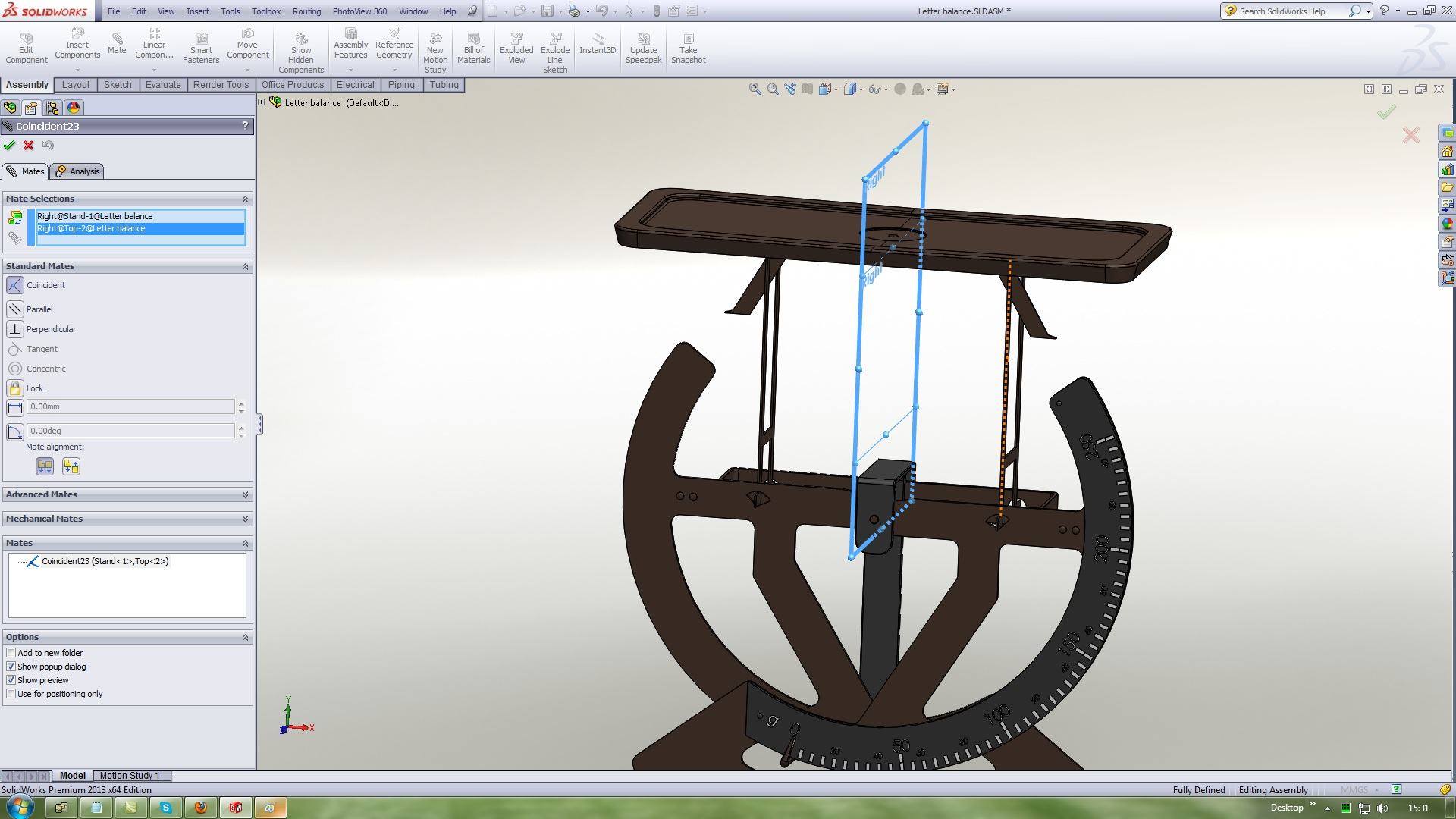37. ### Step 37:

Use Standard mates Tangent and mate this twoo faces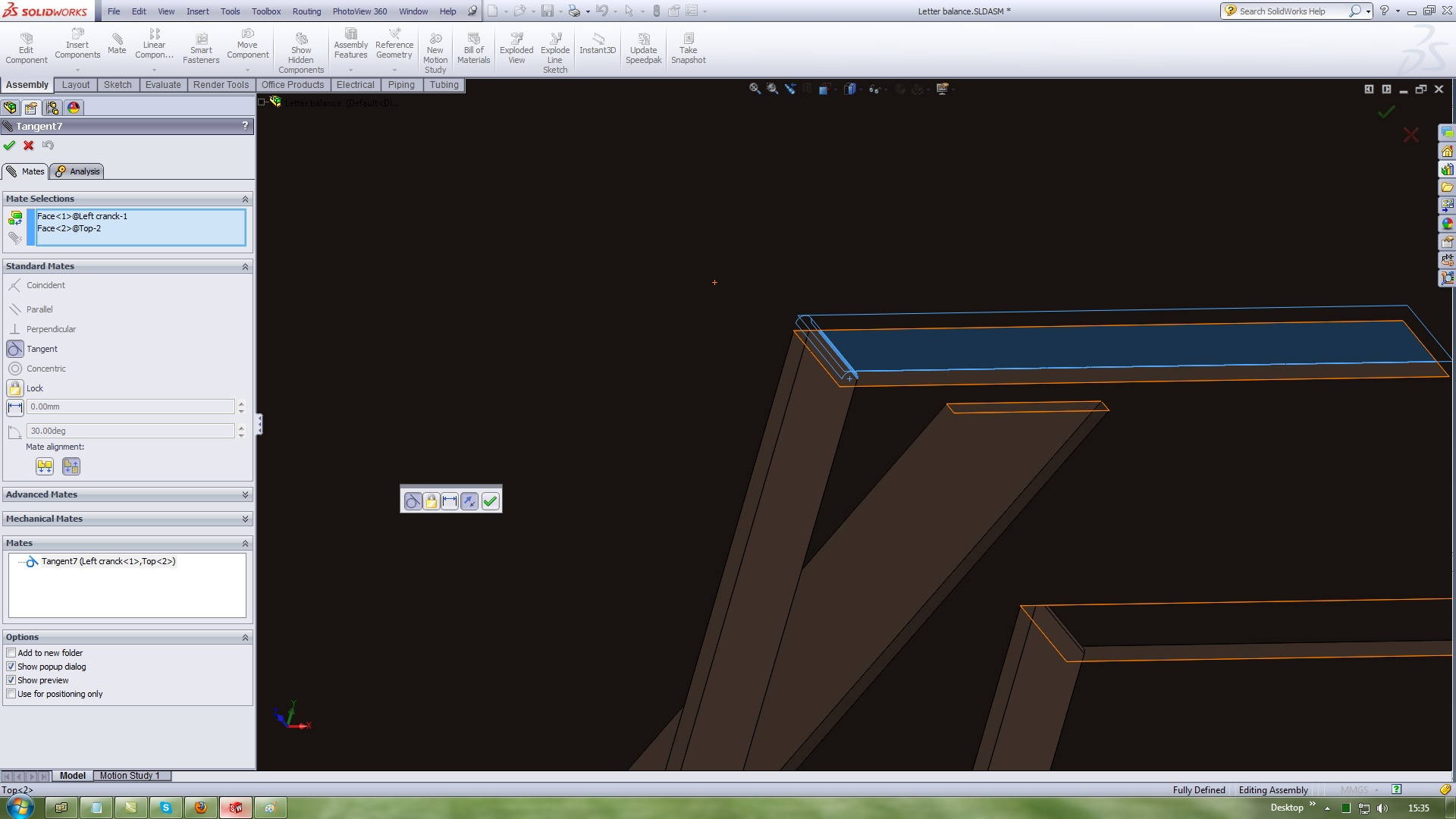38. ### Step 38:

Use Standard mates Tangent and mate this twoo faces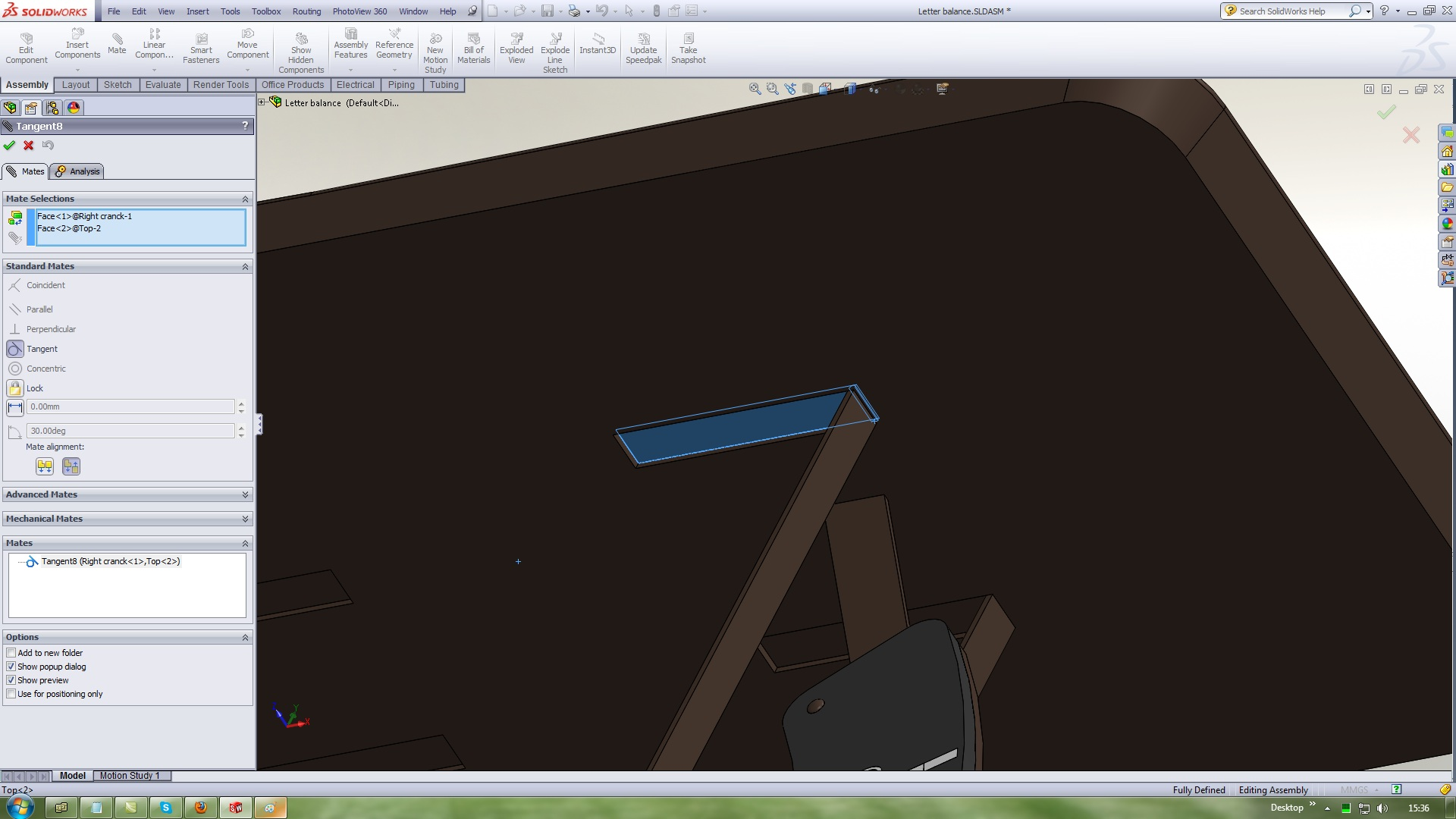39. ### Step 39:

Use Standard mates Coincident and mate this twoo faces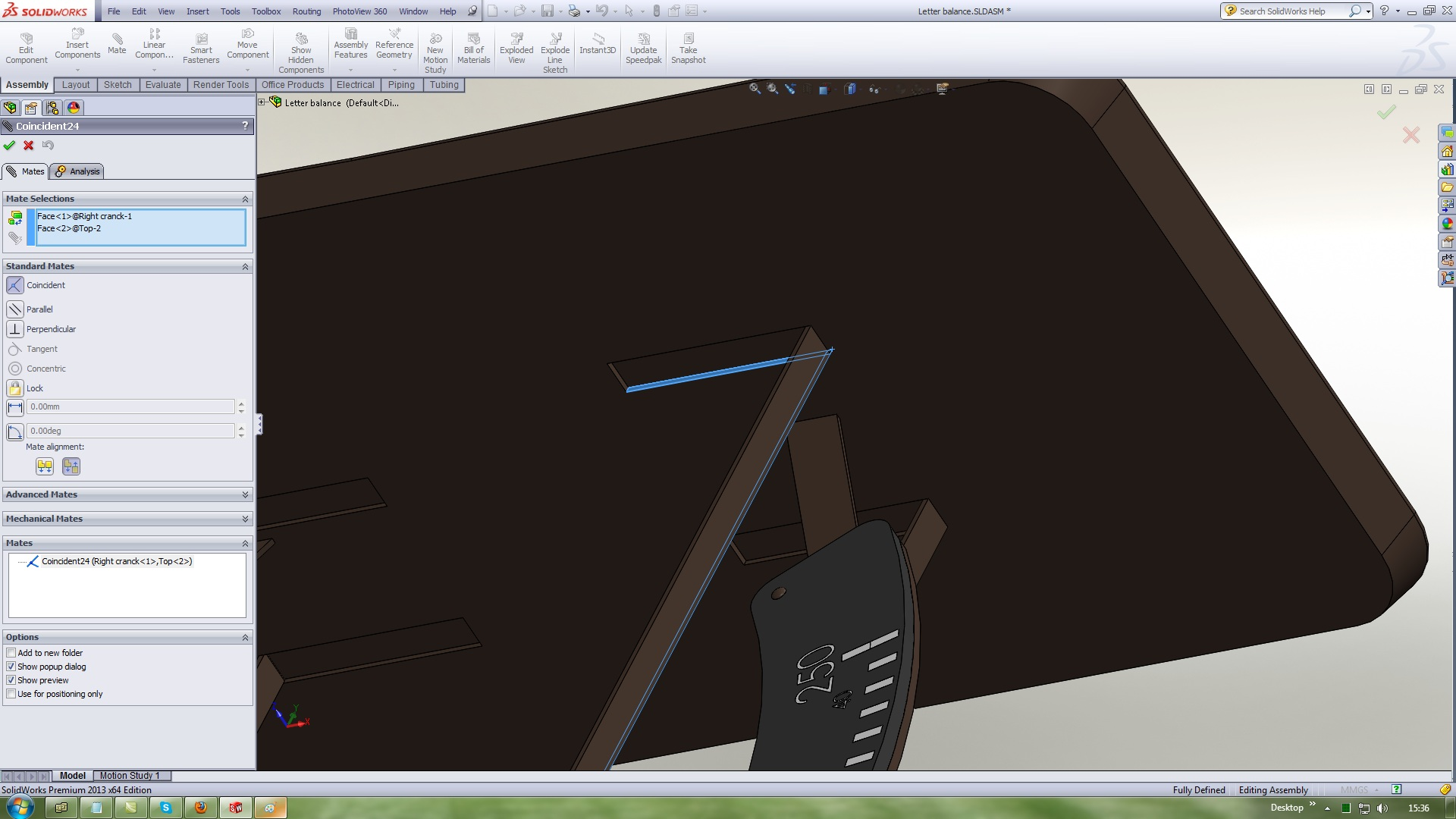40. ### Step 40:

Use Standard mates Coincident and mate this twoo faces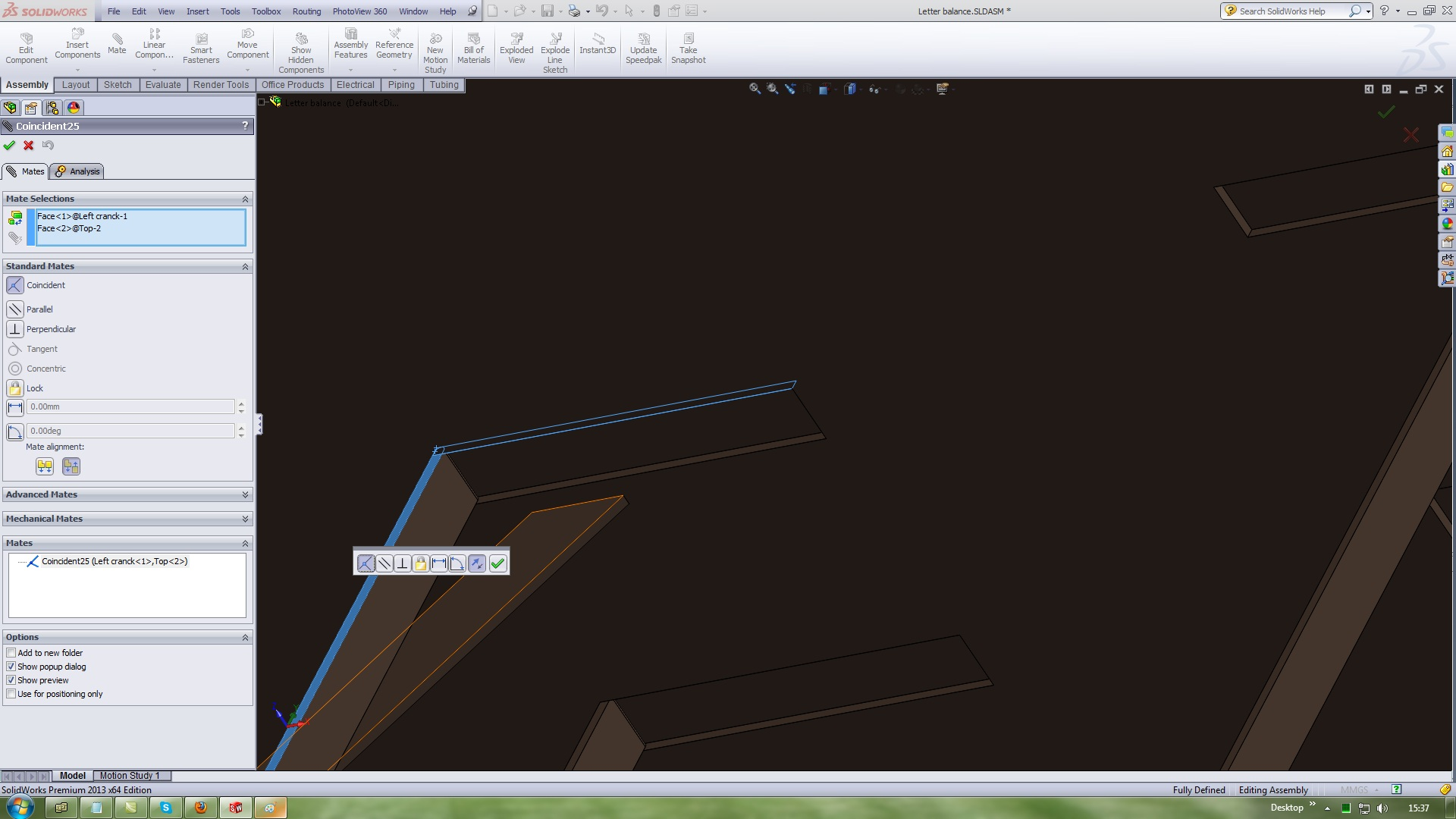41. ### Step 41:

Insert a Upper hinge
Use Advanced mates Width and mate it with this references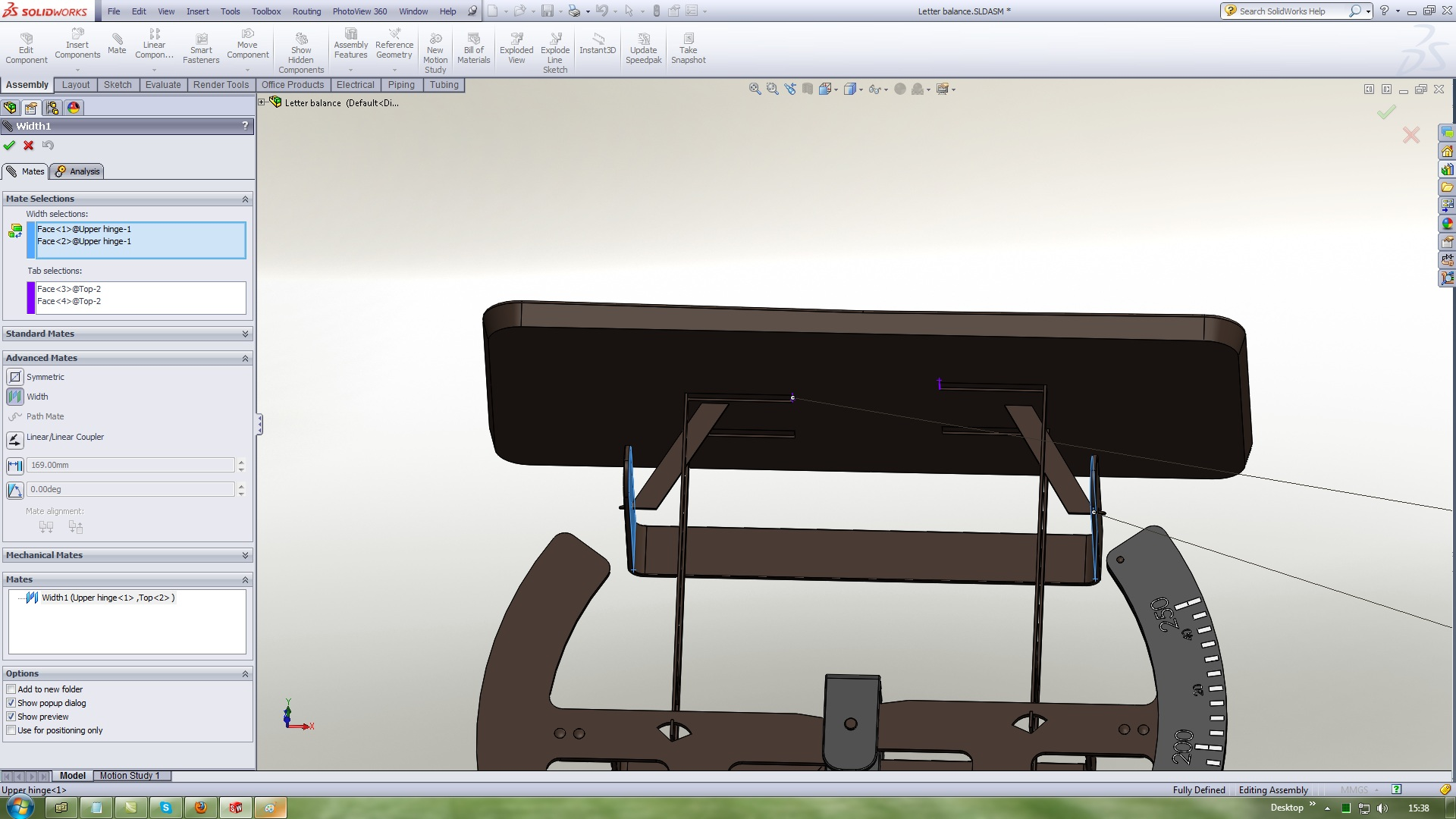42. ### Step 42:

Use Standard mates Distance and mate this twoo faces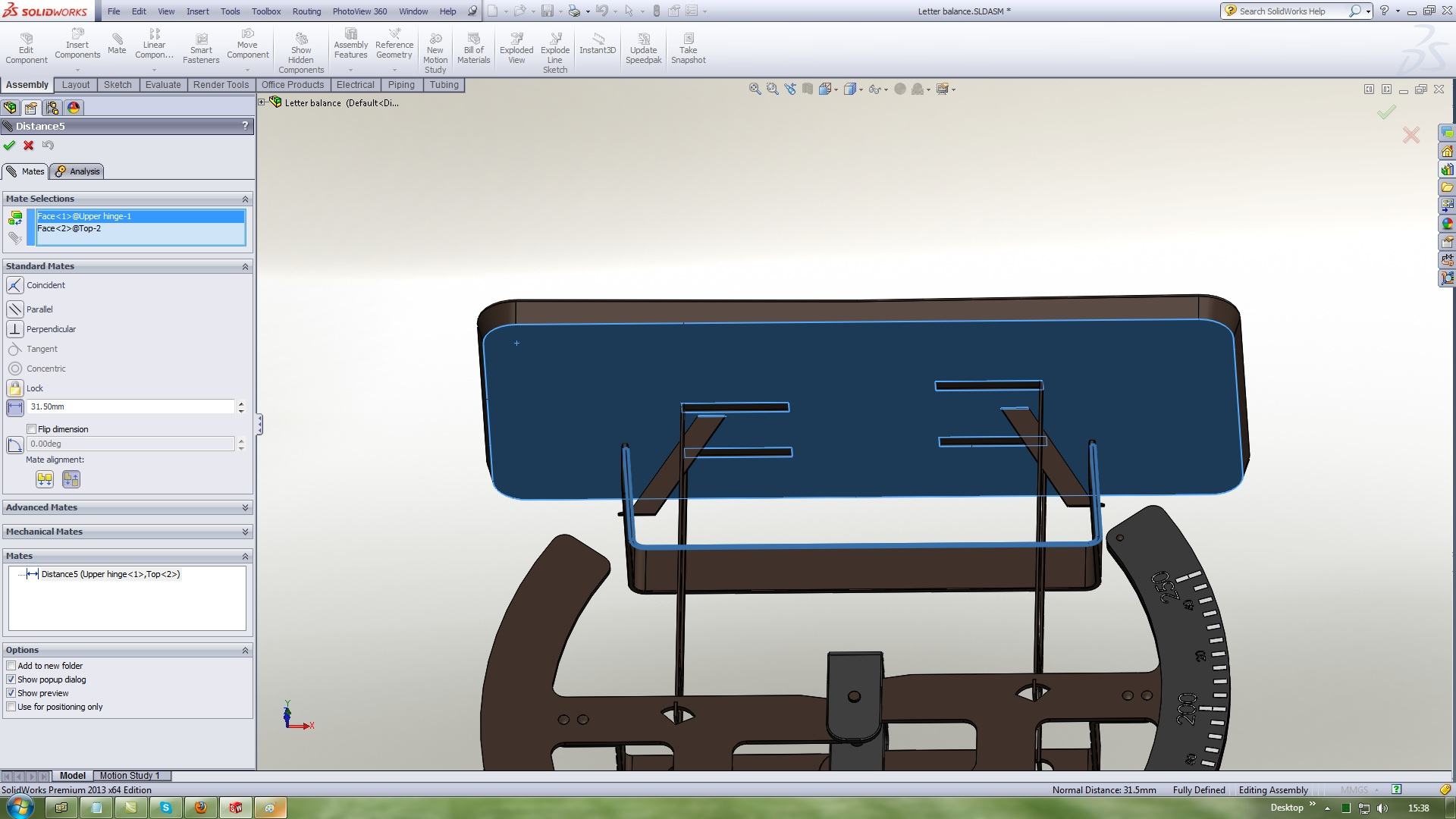43. ### Step 43:

Use Standard mates Distance and mate this twoo faces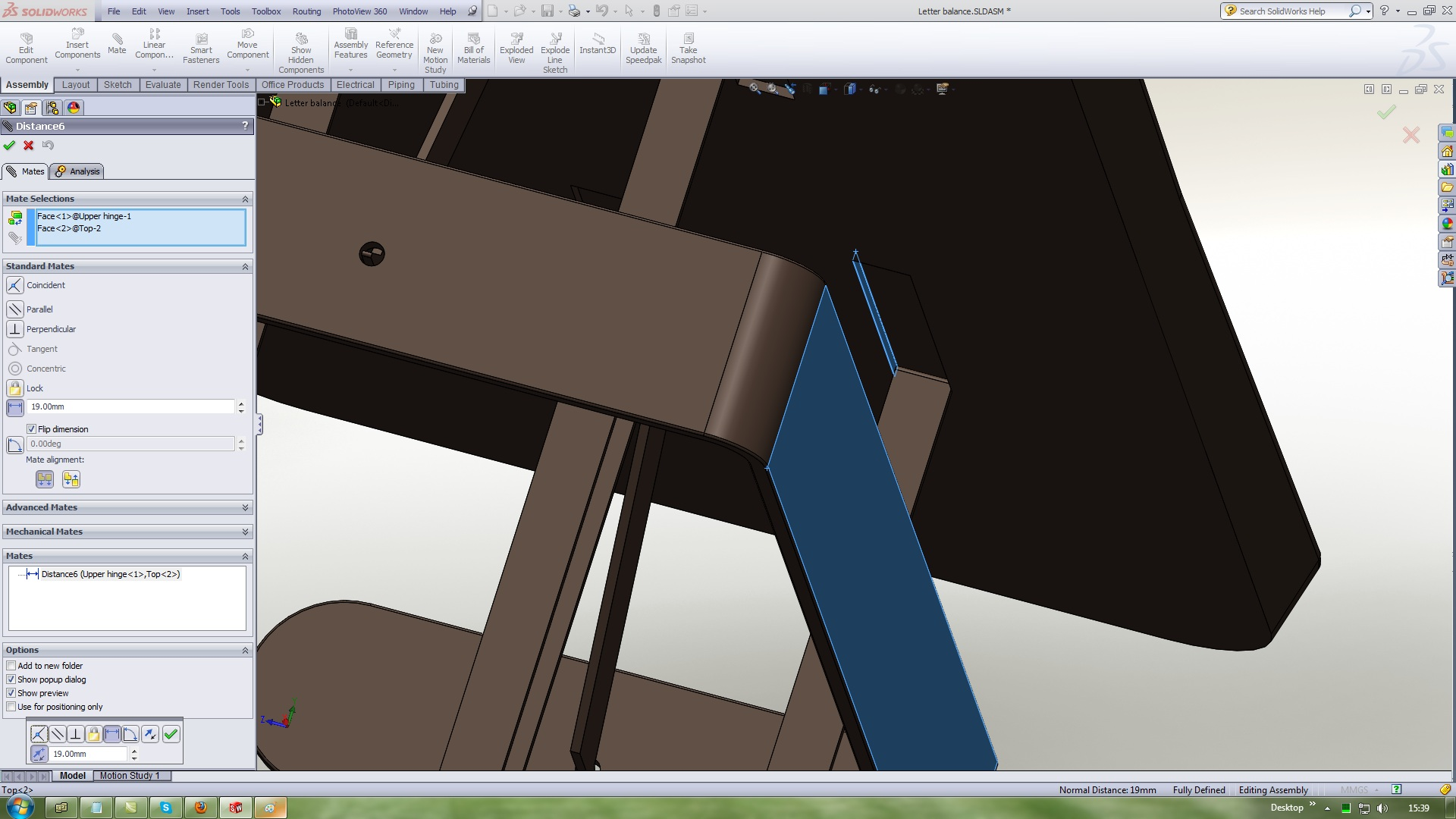44. ### Step 44:

Edit the Stand part and create a new plane with this references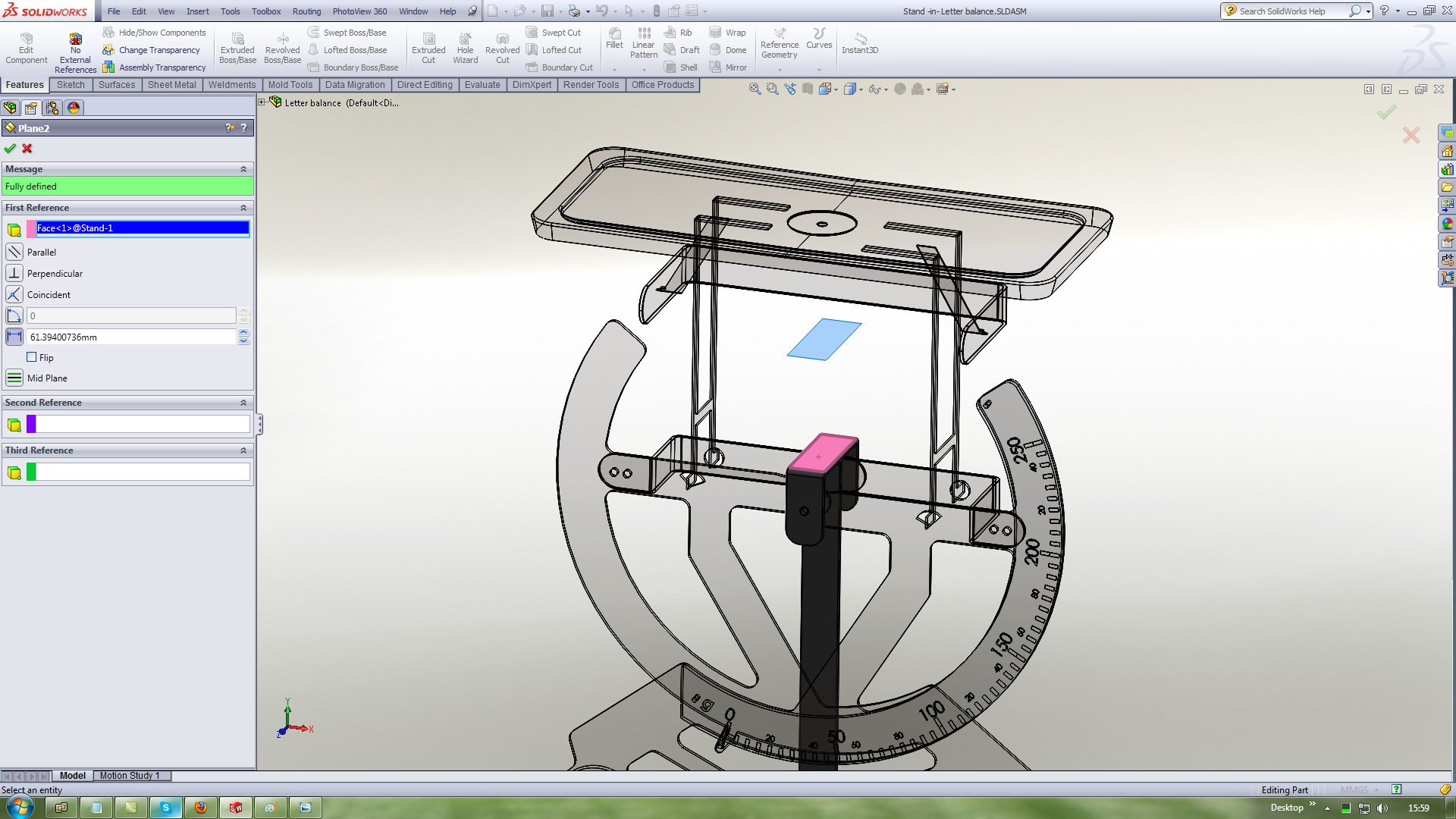45. ### Step 45:

Use Standard mates Limit Distance and mate it with this references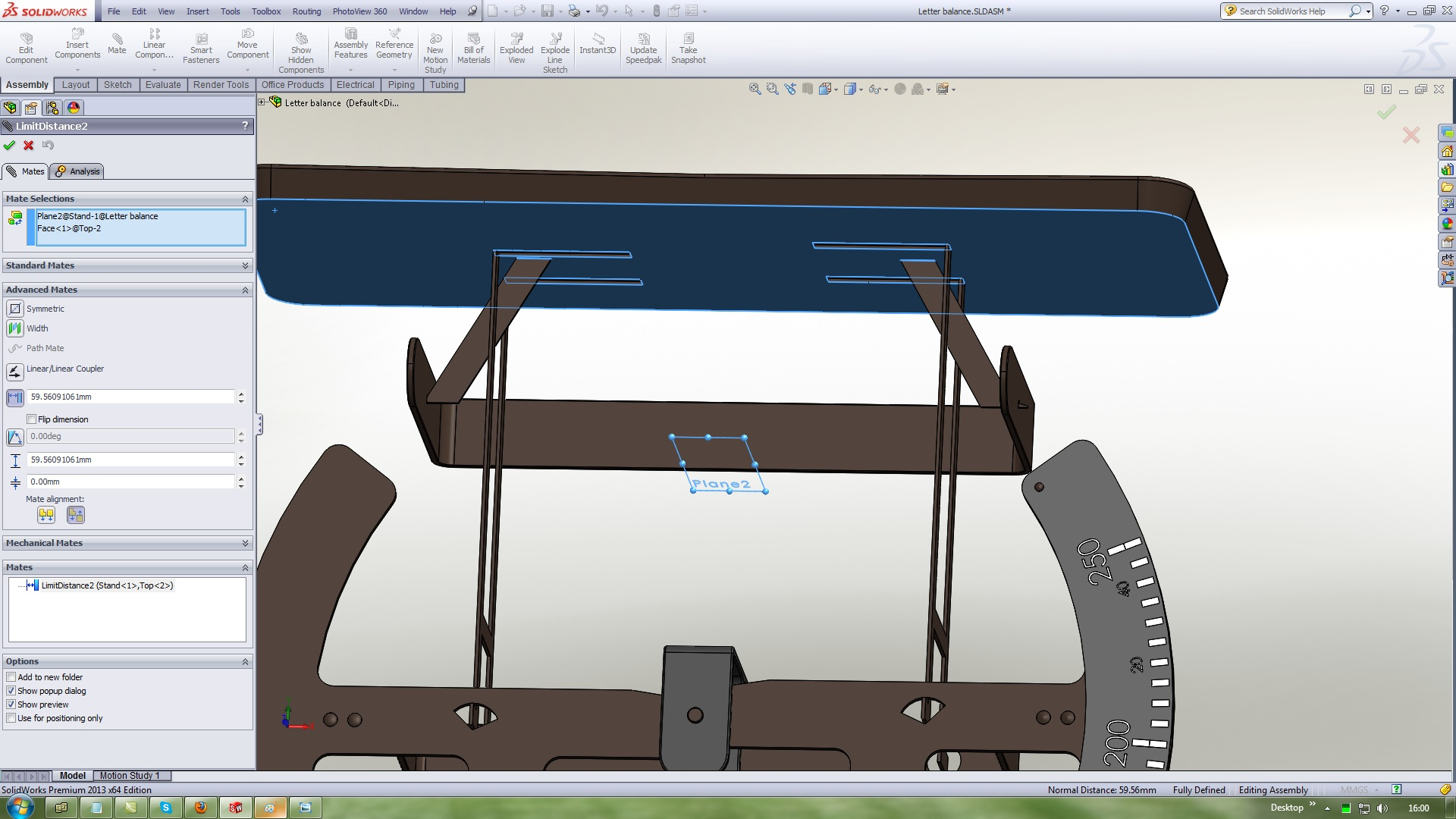46. ### Step 46:

The Assembly must be Fully Defined